Скачать презентацию PID Tuning using the SIMC rules Sigurd Skogestad

a3f305a256db009efbfda2103f2c8f6d.ppt

• Количество слайдов: 78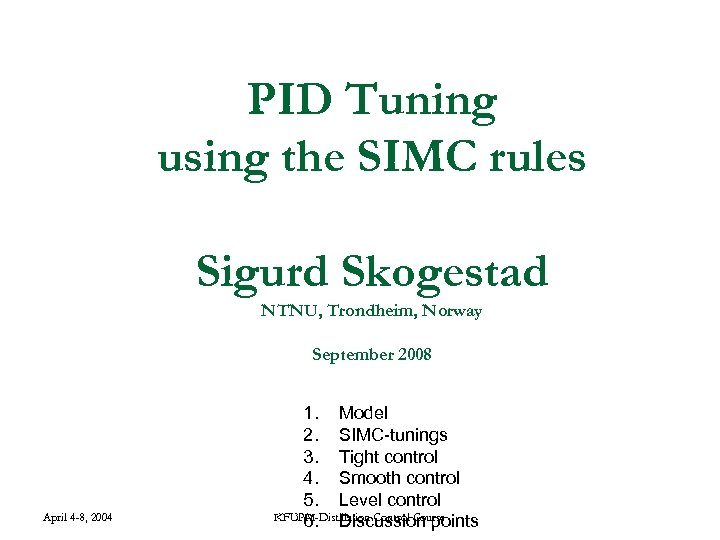PID Tuning using the SIMC rules Sigurd Skogestad NTNU, Trondheim, Norway September 2008 April 4 -8, 2004 1. Model 2. SIMC-tunings 3. Tight control 4. Smooth control 5. Level control KFUPM-Distillation Control Course 6. Discussion points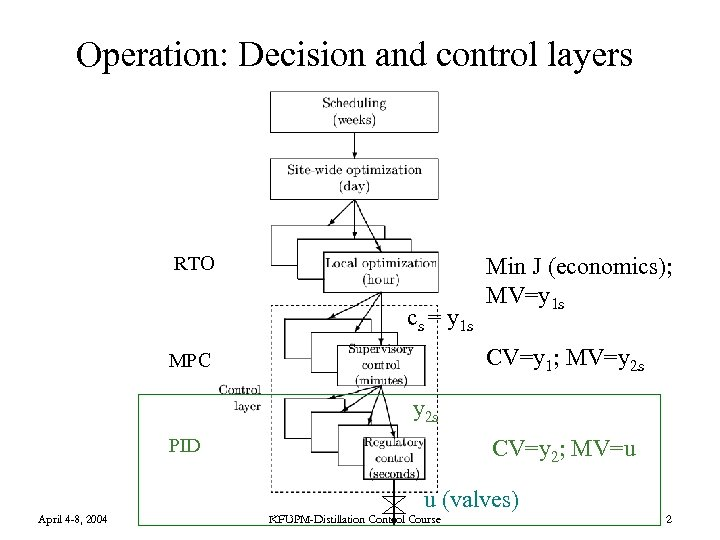Operation: Decision and control layers RTO cs = y 1 s Min J (economics); MV=y 1 s CV=y 1; MV=y 2 s MPC y 2 s PID CV=y 2; MV=u u (valves) April 4 -8, 2004 KFUPM-Distillation Control Course 2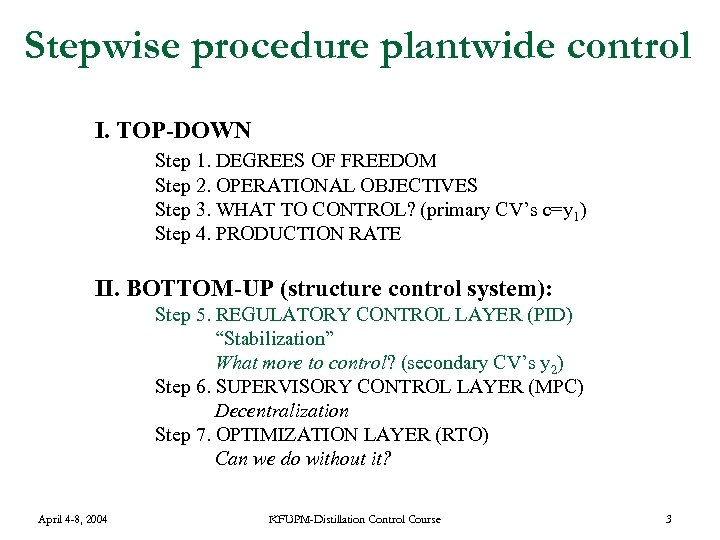Stepwise procedure plantwide control I. TOP-DOWN Step 1. DEGREES OF FREEDOM Step 2. OPERATIONAL OBJECTIVES Step 3. WHAT TO CONTROL? (primary CV’s c=y 1) Step 4. PRODUCTION RATE II. BOTTOM-UP (structure control system): Step 5. REGULATORY CONTROL LAYER (PID) “Stabilization” What more to control? (secondary CV’s y 2) Step 6. SUPERVISORY CONTROL LAYER (MPC) Decentralization Step 7. OPTIMIZATION LAYER (RTO) Can we do without it? April 4 -8, 2004 KFUPM-Distillation Control Course 3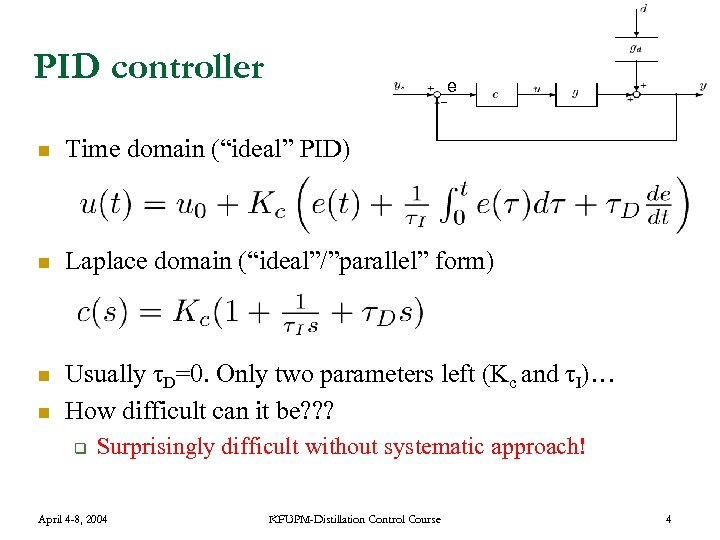PID controller e n Time domain (“ideal” PID) n Laplace domain (“ideal”/”parallel” form) n Usually τD=0. Only two parameters left (Kc and τI)… How difficult can it be? ? ? n q Surprisingly difficult without systematic approach! April 4 -8, 2004 KFUPM-Distillation Control Course 4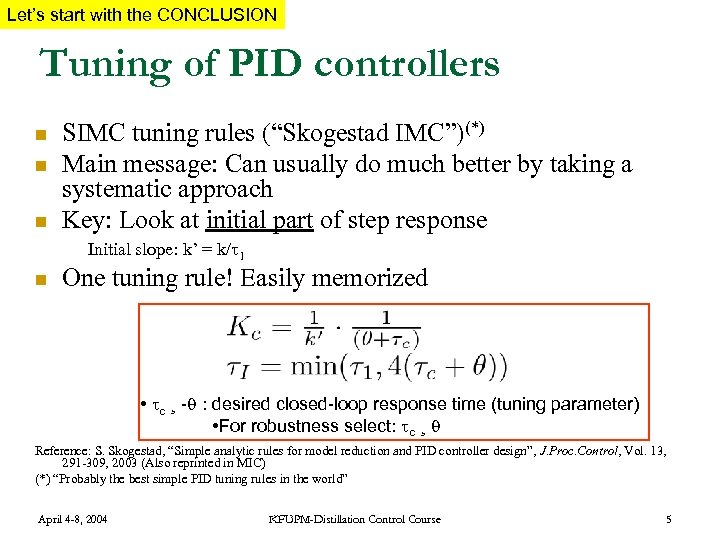Let’s start with the CONCLUSION Tuning of PID controllers n n n SIMC tuning rules (“Skogestad IMC”)(*) Main message: Can usually do much better by taking a systematic approach Key: Look at initial part of step response Initial slope: k’ = k/ 1 n One tuning rule! Easily memorized • c ¸ - : desired closed-loop response time (tuning parameter) • For robustness select: c ¸ Reference: S. Skogestad, “Simple analytic rules for model reduction and PID controller design”, J. Proc. Control, Vol. 13, 291 -309, 2003 (Also reprinted in MIC) (*) “Probably the best simple PID tuning rules in the world” April 4 -8, 2004 KFUPM-Distillation Control Course 5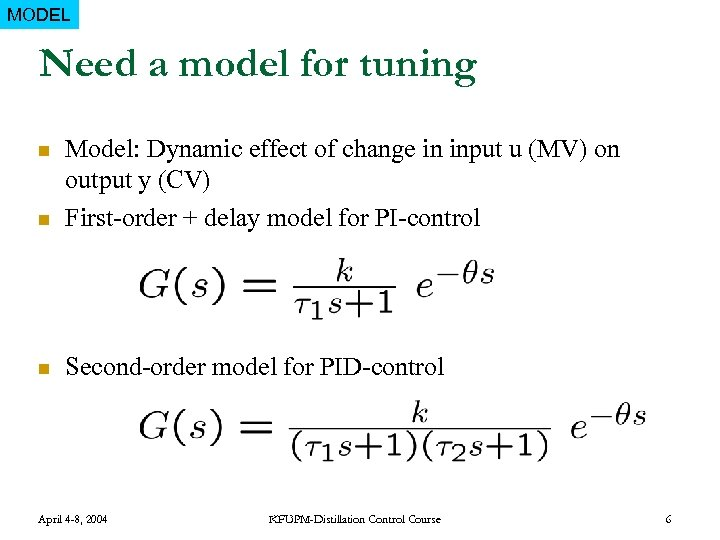MODEL Need a model for tuning n Model: Dynamic effect of change in input u (MV) on output y (CV) First-order + delay model for PI-control n Second-order model for PID-control n April 4 -8, 2004 KFUPM-Distillation Control Course 6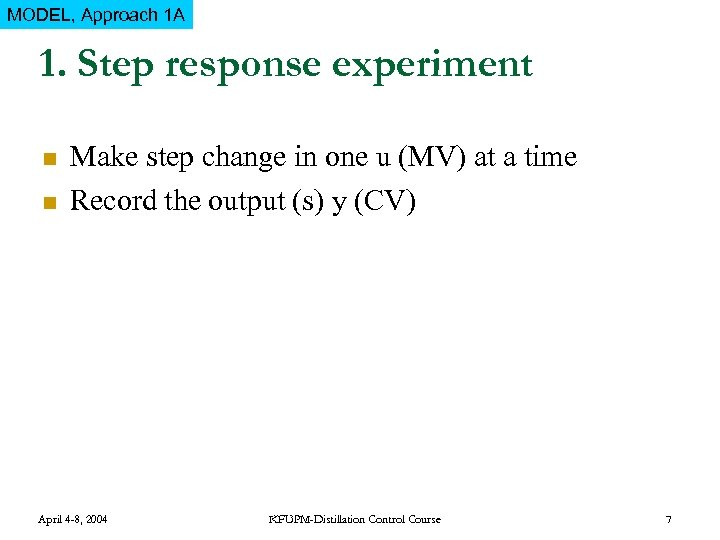MODEL, Approach 1 A 1. Step response experiment n n Make step change in one u (MV) at a time Record the output (s) y (CV) April 4 -8, 2004 KFUPM-Distillation Control Course 7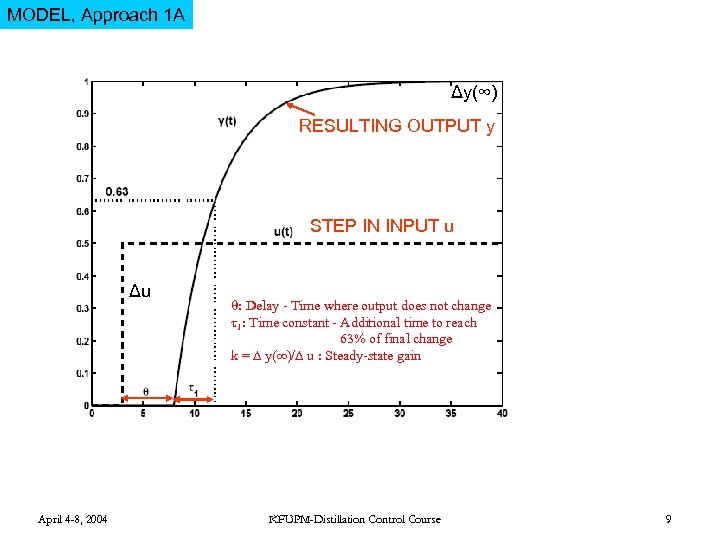MODEL, Approach 1 A Δy(∞) RESULTING OUTPUT y STEP IN INPUT u Δu April 4 -8, 2004 : Delay - Time where output does not change 1: Time constant - Additional time to reach 63% of final change k = y(∞)/ u : Steady-state gain KFUPM-Distillation Control Course 9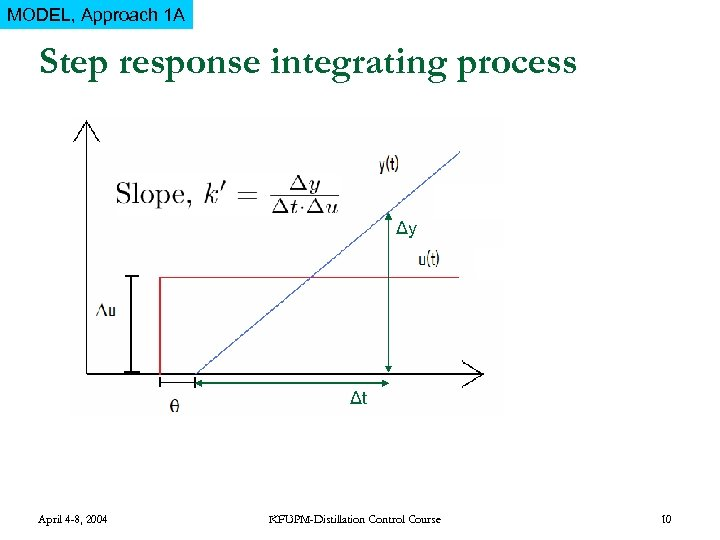MODEL, Approach 1 A Step response integrating process Δy Δt April 4 -8, 2004 KFUPM-Distillation Control Course 10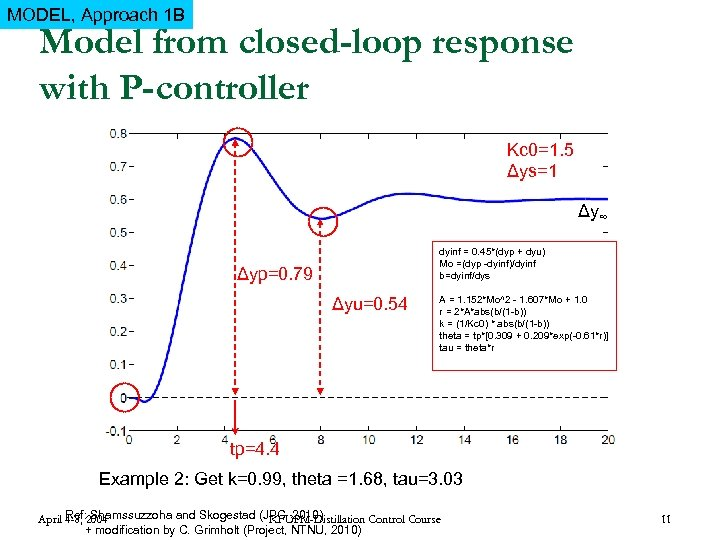MODEL, Approach 1 B Model from closed-loop response with P-controller Kc 0=1. 5 Δys=1 Δy∞ dyinf = 0. 45*(dyp + dyu) Mo =(dyp -dyinf)/dyinf b=dyinf/dys Δyp=0. 79 Δyu=0. 54 A = 1. 152*Mo^2 - 1. 607*Mo + 1. 0 r = 2*A*abs(b/(1 -b)) k = (1/Kc 0) * abs(b/(1 -b)) theta = tp*[0. 309 + 0. 209*exp(-0. 61*r)] tau = theta*r tp=4. 4 Example 2: Get k=0. 99, theta =1. 68, tau=3. 03 Ref: Shamssuzzoha and Skogestad (JPC, 2010) April 4 -8, 2004 KFUPM-Distillation Control Course + modification by C. Grimholt (Project, NTNU, 2010) 11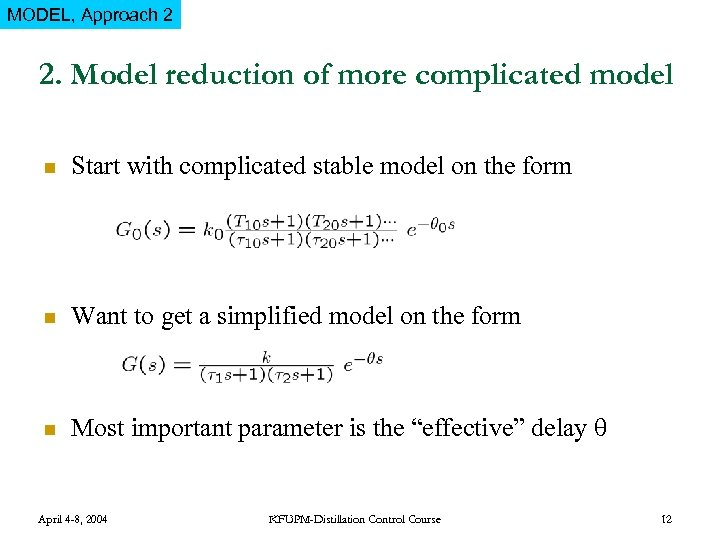MODEL, Approach 2 2. Model reduction of more complicated model n Start with complicated stable model on the form n Want to get a simplified model on the form n Most important parameter is the “effective” delay April 4 -8, 2004 KFUPM-Distillation Control Course 12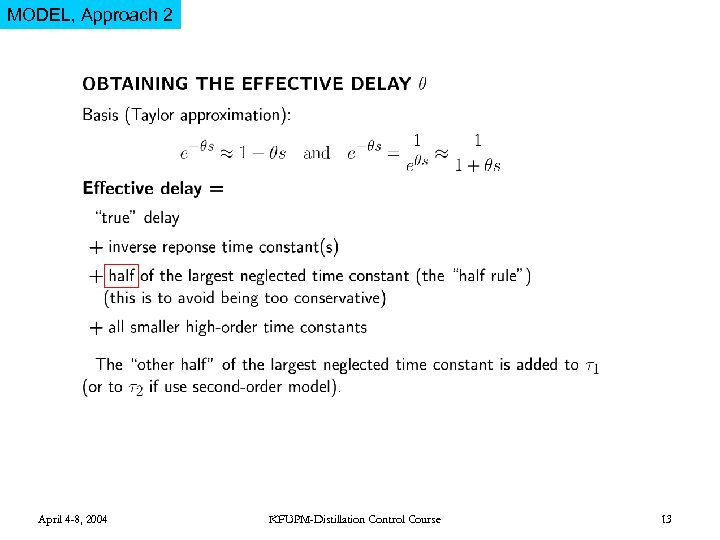MODEL, Approach 2 April 4 -8, 2004 KFUPM-Distillation Control Course 13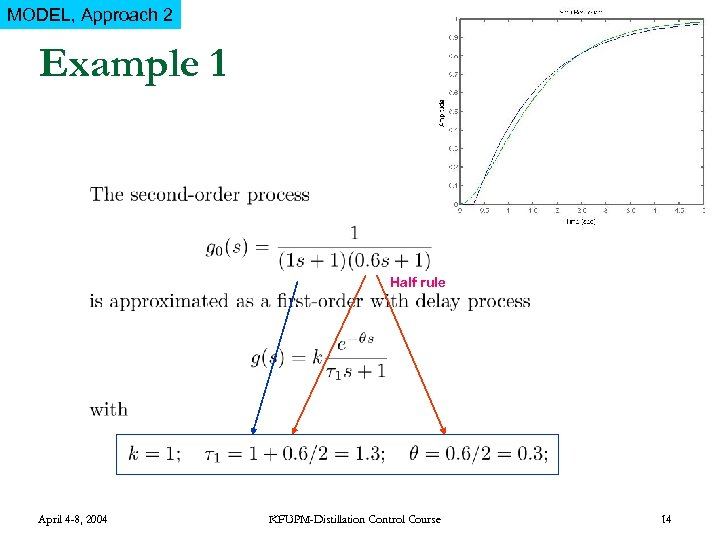MODEL, Approach 2 Example 1 Half rule April 4 -8, 2004 KFUPM-Distillation Control Course 14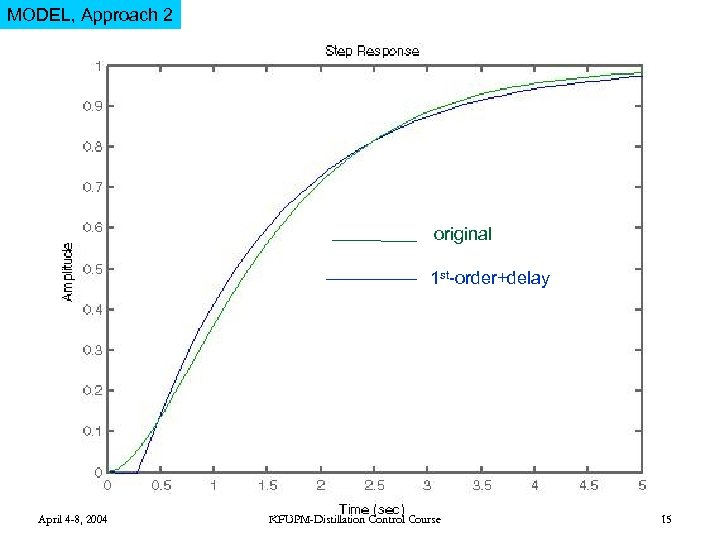MODEL, Approach 2 original 1 st-order+delay April 4 -8, 2004 KFUPM-Distillation Control Course 15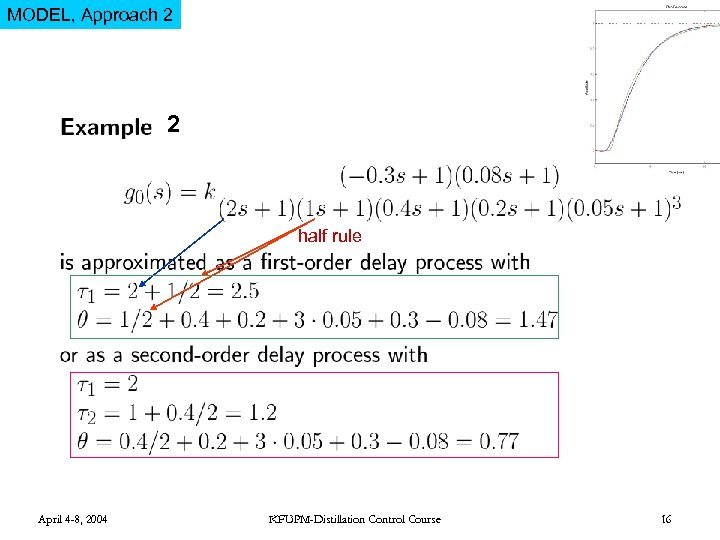MODEL, Approach 2 2 half rule April 4 -8, 2004 KFUPM-Distillation Control Course 16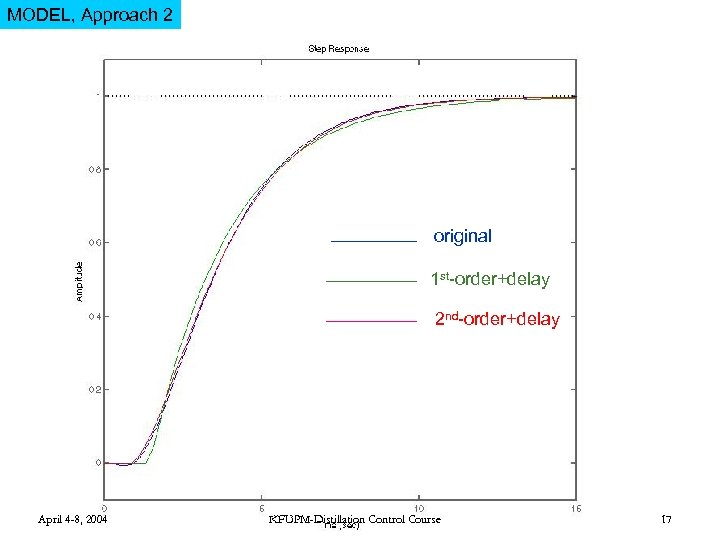MODEL, Approach 2 original 1 st-order+delay 2 nd-order+delay April 4 -8, 2004 KFUPM-Distillation Control Course 17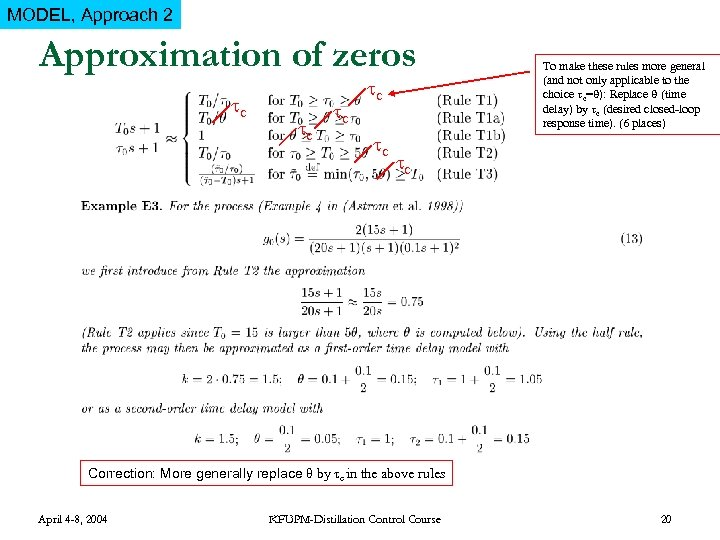MODEL, Approach 2 Approximation of zeros c c c To make these rules more general (and not only applicable to the choice c= ): Replace (time delay) by c (desired closed-loop response time). (6 places) c Correction: More generally replace θ by τc in the above rules April 4 -8, 2004 KFUPM-Distillation Control Course 20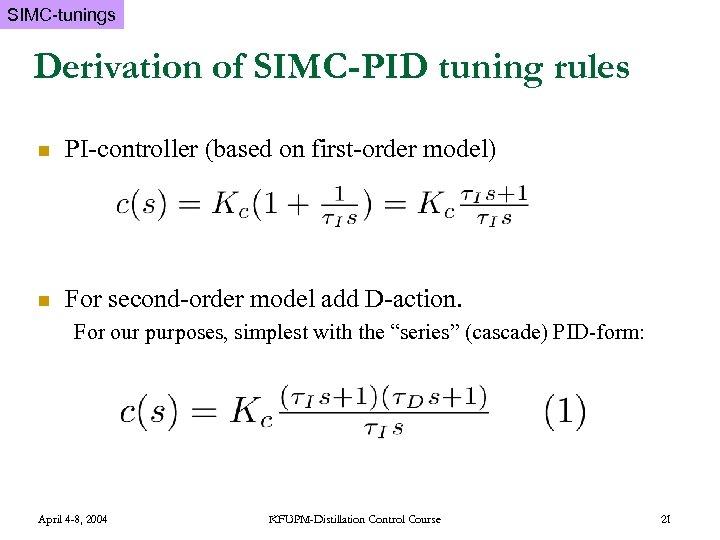SIMC-tunings Derivation of SIMC-PID tuning rules n PI-controller (based on first-order model) n For second-order model add D-action. For our purposes, simplest with the “series” (cascade) PID-form: April 4 -8, 2004 KFUPM-Distillation Control Course 21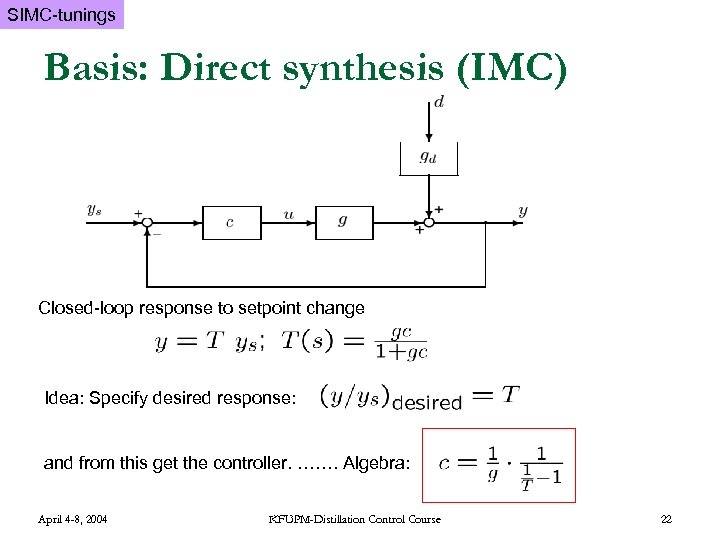SIMC-tunings Basis: Direct synthesis (IMC) Closed-loop response to setpoint change Idea: Specify desired response: and from this get the controller. ……. Algebra: April 4 -8, 2004 KFUPM-Distillation Control Course 22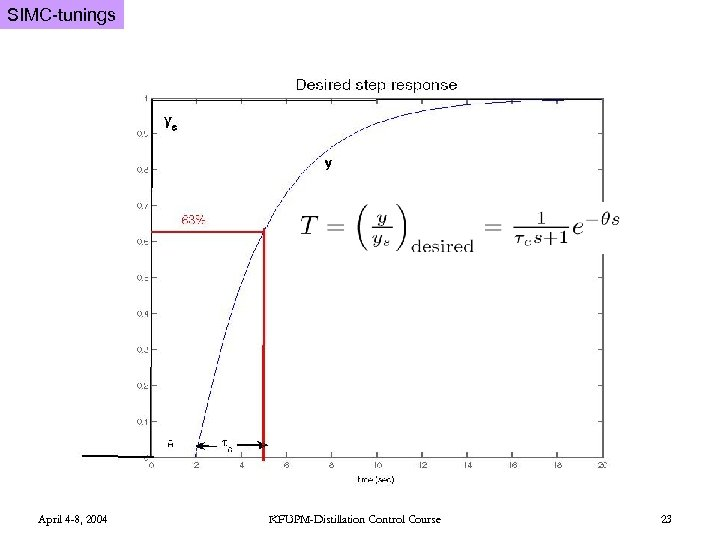SIMC-tunings April 4 -8, 2004 KFUPM-Distillation Control Course 23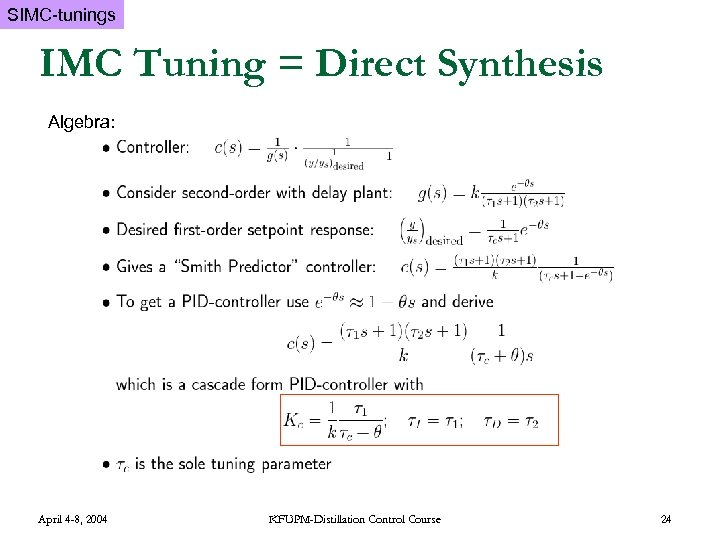SIMC-tunings IMC Tuning = Direct Synthesis Algebra: April 4 -8, 2004 KFUPM-Distillation Control Course 24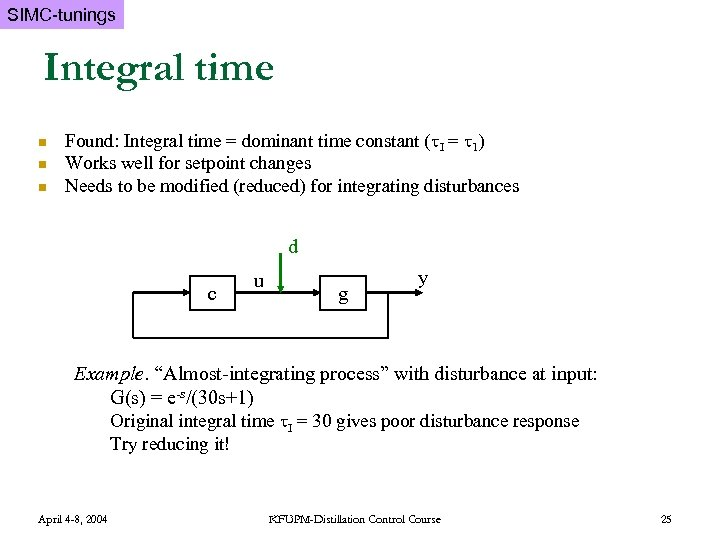SIMC-tunings Integral time n n n Found: Integral time = dominant time constant ( I = 1) Works well for setpoint changes Needs to be modified (reduced) for integrating disturbances d c u g y Example. “Almost-integrating process” with disturbance at input: G(s) = e-s/(30 s+1) Original integral time I = 30 gives poor disturbance response Try reducing it! April 4 -8, 2004 KFUPM-Distillation Control Course 25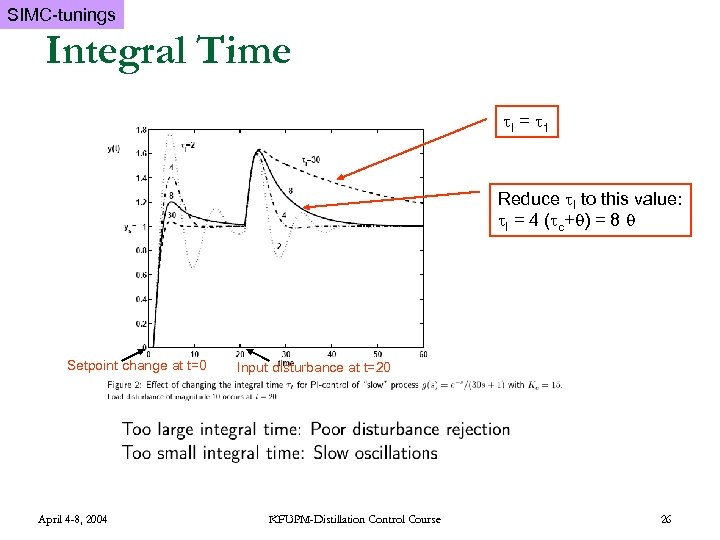SIMC-tunings Integral Time I = 1 Reduce I to this value: I = 4 ( c+ ) = 8 Setpoint change at t=0 April 4 -8, 2004 Input disturbance at t=20 KFUPM-Distillation Control Course 26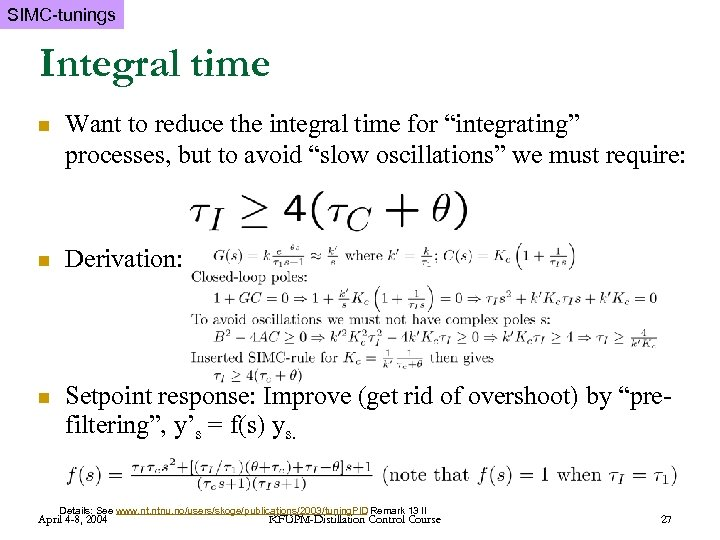SIMC-tunings Integral time n Want to reduce the integral time for “integrating” processes, but to avoid “slow oscillations” we must require: n Derivation: n Setpoint response: Improve (get rid of overshoot) by “prefiltering”, y’s = f(s) ys. Details: See www. ntnu. no/users/skoge/publications/2003/tuning. PID Remark 13 II April 4 -8, 2004 KFUPM-Distillation Control Course 27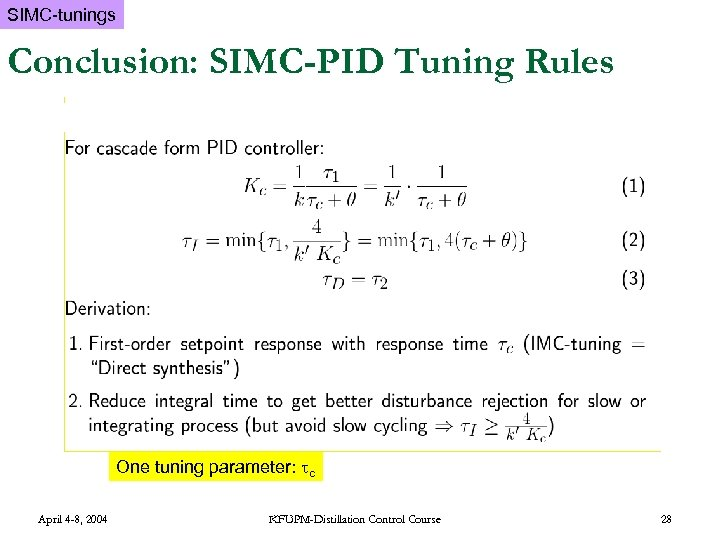SIMC-tunings Conclusion: SIMC-PID Tuning Rules One tuning parameter: c April 4 -8, 2004 KFUPM-Distillation Control Course 28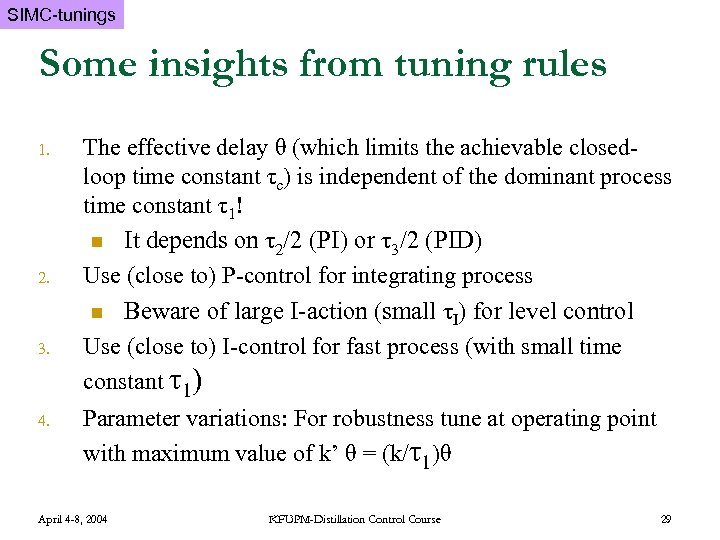SIMC-tunings Some insights from tuning rules 1. 2. 3. 4. The effective delay θ (which limits the achievable closedloop time constant τc) is independent of the dominant process time constant τ1! n It depends on τ2/2 (PI) or τ3/2 (PID) Use (close to) P-control for integrating process n Beware of large I-action (small τI) for level control Use (close to) I-control for fast process (with small time constant τ1) Parameter variations: For robustness tune at operating point with maximum value of k’ θ = (k/τ1)θ April 4 -8, 2004 KFUPM-Distillation Control Course 29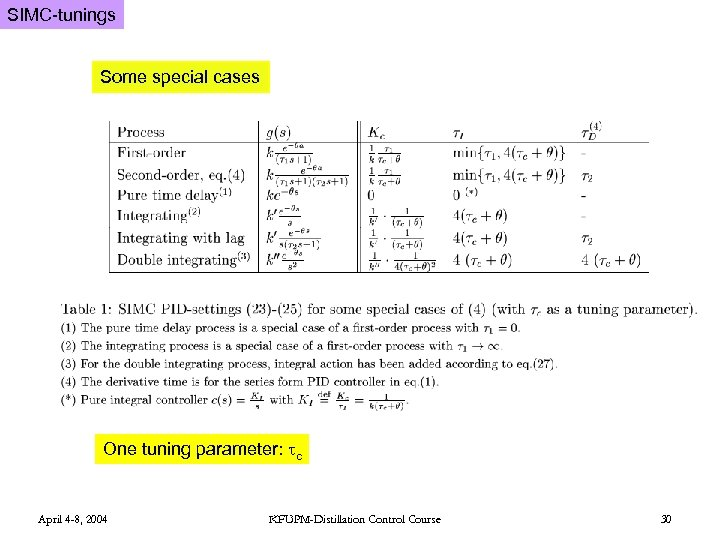SIMC-tunings Some special cases One tuning parameter: c April 4 -8, 2004 KFUPM-Distillation Control Course 30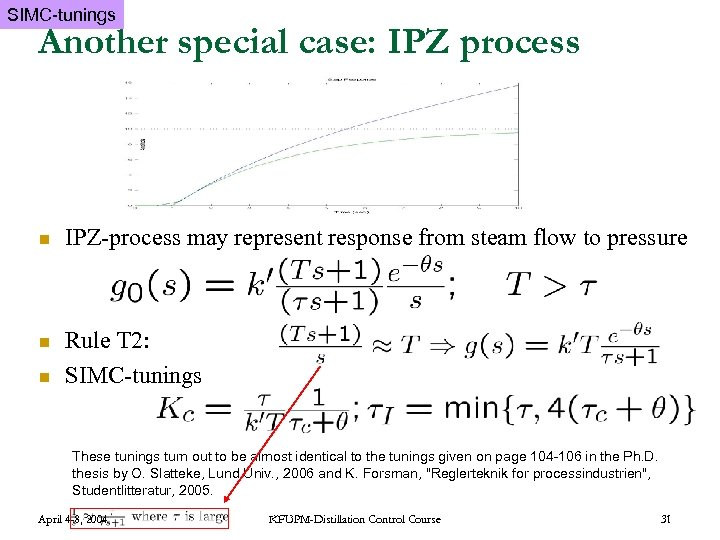SIMC-tunings Another special case: IPZ process n IPZ-process may represent response from steam flow to pressure n Rule T 2: SIMC-tunings n These tunings turn out to be almost identical to the tunings given on page 104 -106 in the Ph. D. thesis by O. Slatteke, Lund Univ. , 2006 and K. Forsman, "Reglerteknik for processindustrien", Studentlitteratur, 2005. April 4 -8, 2004 KFUPM-Distillation Control Course 31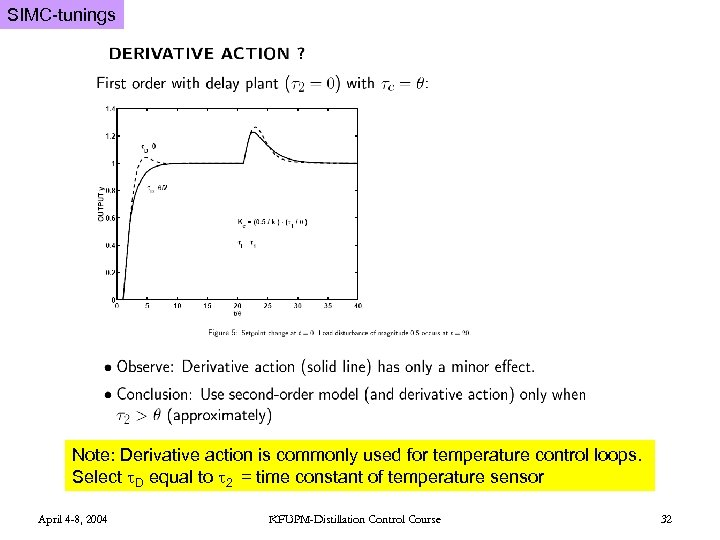SIMC-tunings Note: Derivative action is commonly used for temperature control loops. Select D equal to 2 = time constant of temperature sensor April 4 -8, 2004 KFUPM-Distillation Control Course 32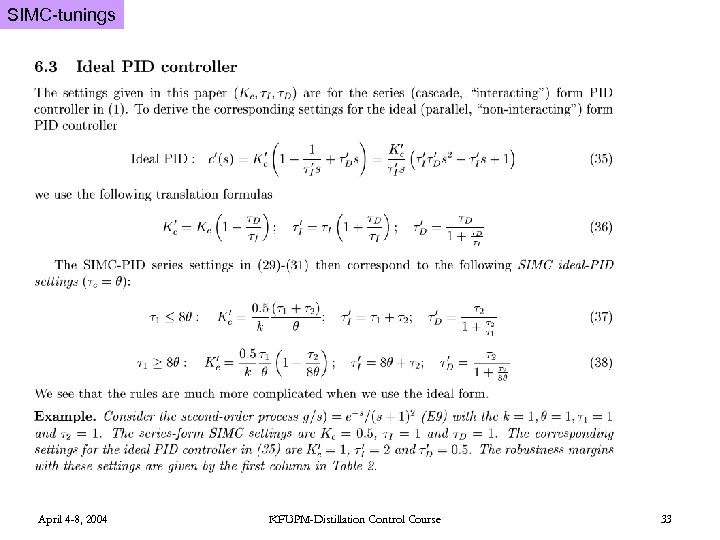SIMC-tunings April 4 -8, 2004 KFUPM-Distillation Control Course 33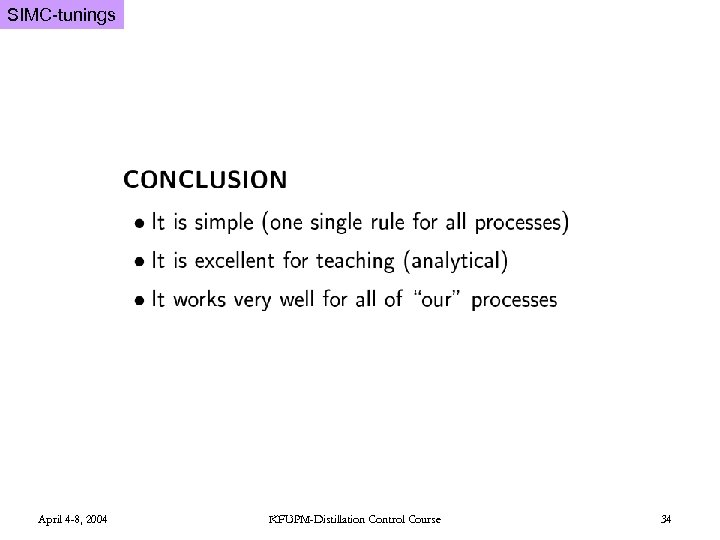SIMC-tunings April 4 -8, 2004 KFUPM-Distillation Control Course 34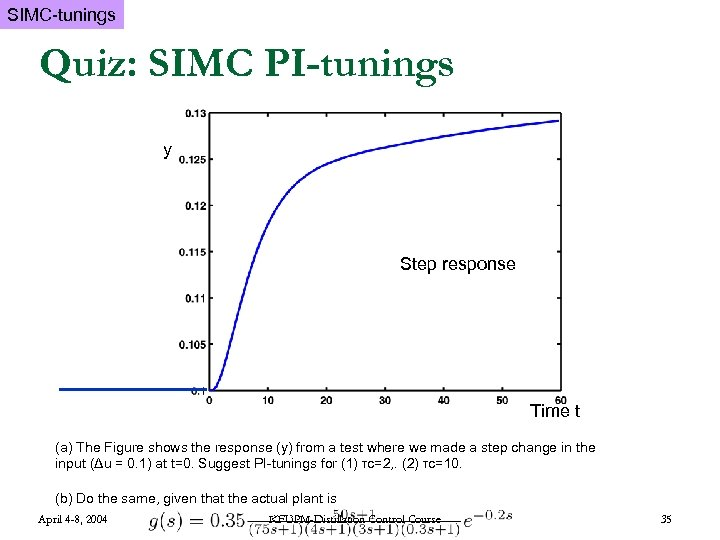SIMC-tunings Quiz: SIMC PI-tunings y y Step response t [s]Time t (a) The Figure shows the response (y) from a test where we made a step change in the input (Δu = 0. 1) at t=0. Suggest PI-tunings for (1) τc=2, . (2) τc=10. (b) Do the same, given that the actual plant is April 4 -8, 2004 KFUPM-Distillation Control Course 35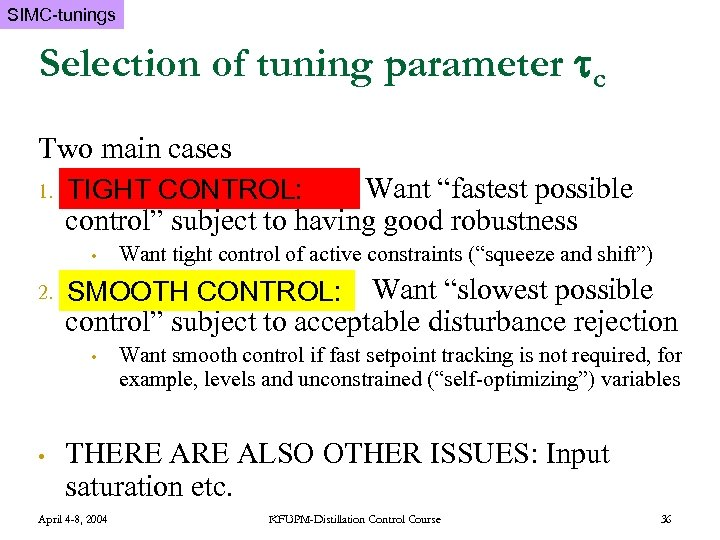SIMC-tunings Selection of tuning parameter c Two main cases 1. TIGHT CONTROL: Want “fastest possible TIGHT CONTROL: control” subject to having good robustness • 2. SMOOTH CONTROL: Want “slowest possible CONTROL: control” subject to acceptable disturbance rejection • • Want tight control of active constraints (“squeeze and shift”) Want smooth control if fast setpoint tracking is not required, for example, levels and unconstrained (“self-optimizing”) variables THERE ALSO OTHER ISSUES: Input saturation etc. April 4 -8, 2004 KFUPM-Distillation Control Course 36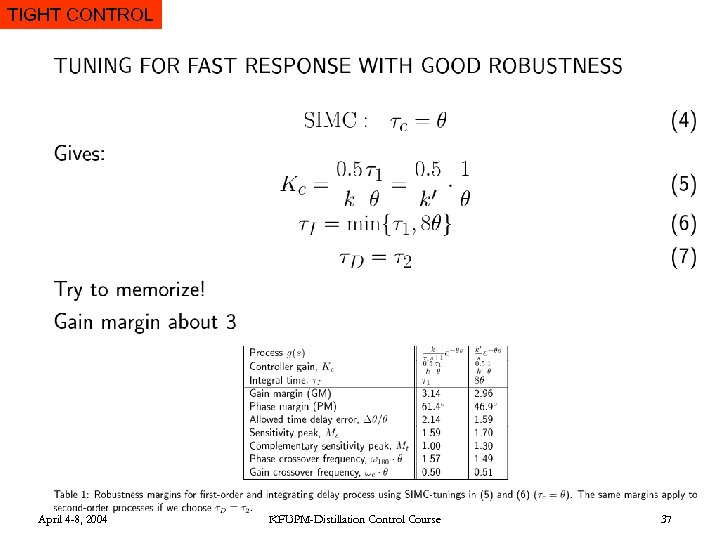TIGHT CONTROL April 4 -8, 2004 KFUPM-Distillation Control Course 37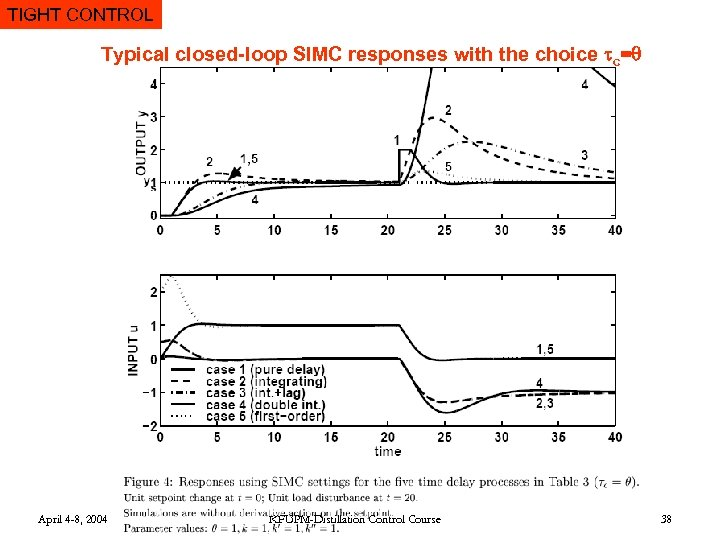TIGHT CONTROL Typical closed-loop SIMC responses with the choice c= April 4 -8, 2004 KFUPM-Distillation Control Course 38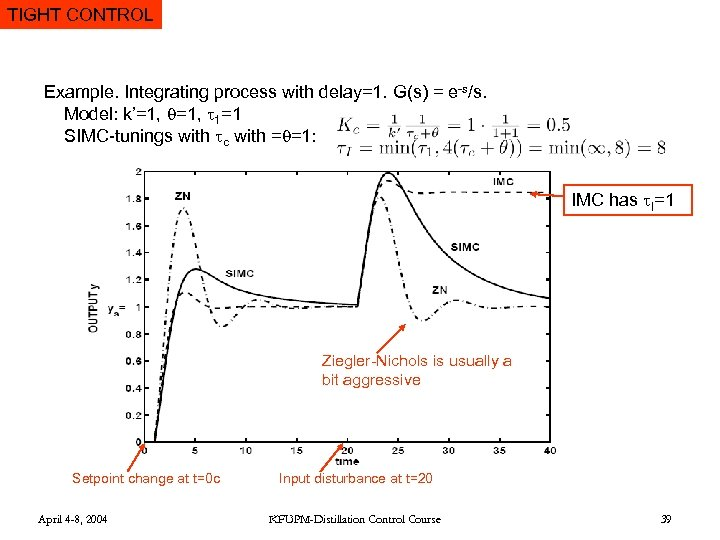TIGHT CONTROL Example. Integrating process with delay=1. G(s) = e-s/s. Model: k’=1, 1=1 SIMC-tunings with c with = =1: IMC has I=1 Ziegler-Nichols is usually a bit aggressive Setpoint change at t=0 c April 4 -8, 2004 Input disturbance at t=20 KFUPM-Distillation Control Course 39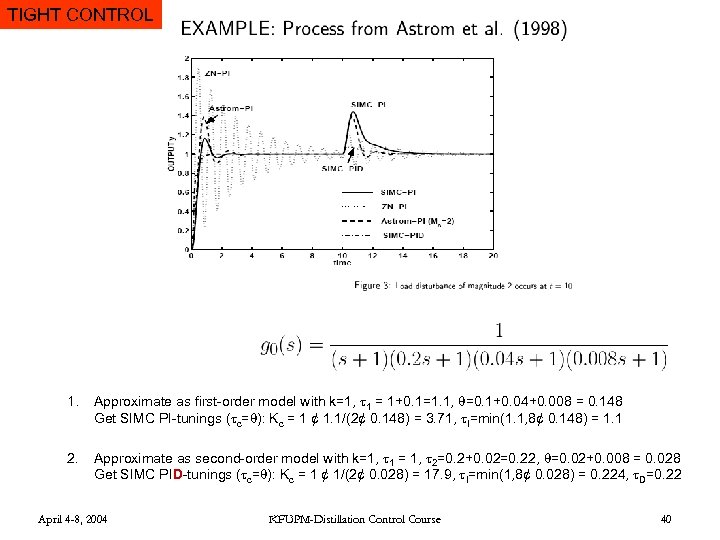TIGHT CONTROL 1. Approximate as first-order model with k=1, 1 = 1+0. 1=1. 1, =0. 1+0. 04+0. 008 = 0. 148 Get SIMC PI-tunings ( c= ): Kc = 1 ¢ 1. 1/(2¢ 0. 148) = 3. 71, I=min(1. 1, 8¢ 0. 148) = 1. 1 2. Approximate as second-order model with k=1, 1 = 1, 2=0. 2+0. 02=0. 22, =0. 02+0. 008 = 0. 028 Get SIMC PID-tunings ( c= ): Kc = 1 ¢ 1/(2¢ 0. 028) = 17. 9, I=min(1, 8¢ 0. 028) = 0. 224, D=0. 22 April 4 -8, 2004 KFUPM-Distillation Control Course 40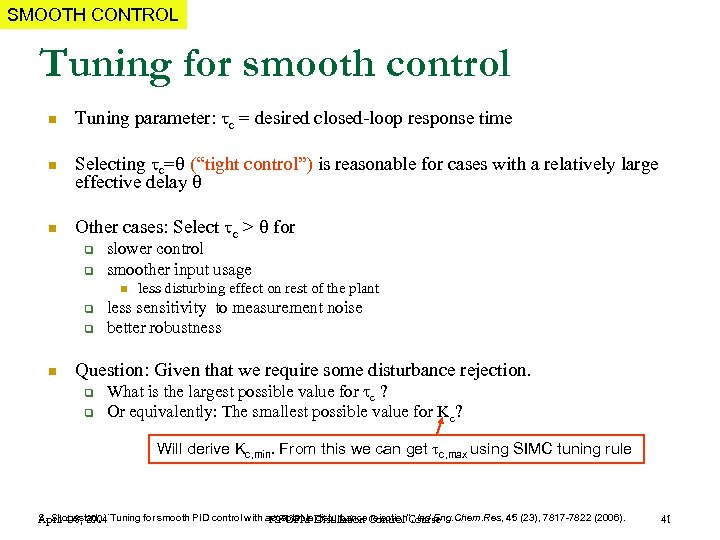SMOOTH CONTROL Tuning for smooth control n Tuning parameter: c = desired closed-loop response time n Selecting c= (“tight control”) is reasonable for cases with a relatively large effective delay n Other cases: Select c > for q q slower control smoother input usage n q q n less disturbing effect on rest of the plant less sensitivity to measurement noise better robustness Question: Given that we require some disturbance rejection. q q What is the largest possible value for c ? Or equivalently: The smallest possible value for Kc? Will derive Kc, min. From this we can get c, max using SIMC tuning rule S. Skogestad, ``Tuning for smooth PID control with acceptable disturbance Control Course April 4 -8, 2004 KFUPM-Distillation rejection'', Ind. Eng. Chem. Res, 45 (23), 7817 -7822 (2006). 41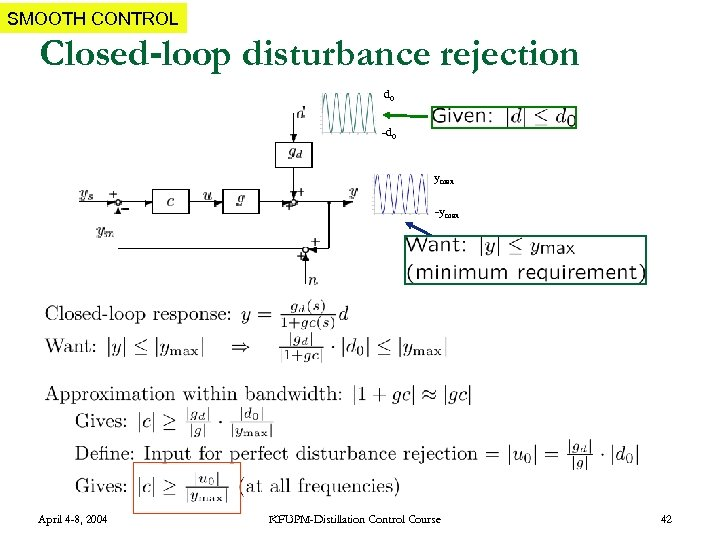SMOOTH CONTROL Closed-loop disturbance rejection d 0 -d 0 ymax -ymax April 4 -8, 2004 KFUPM-Distillation Control Course 42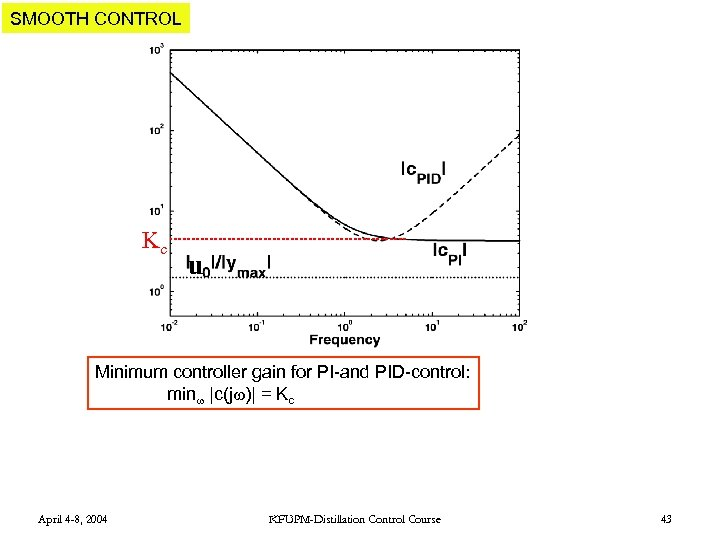SMOOTH CONTROL Kc u Minimum controller gain for PI-and PID-control: min |c(j )| = Kc April 4 -8, 2004 KFUPM-Distillation Control Course 43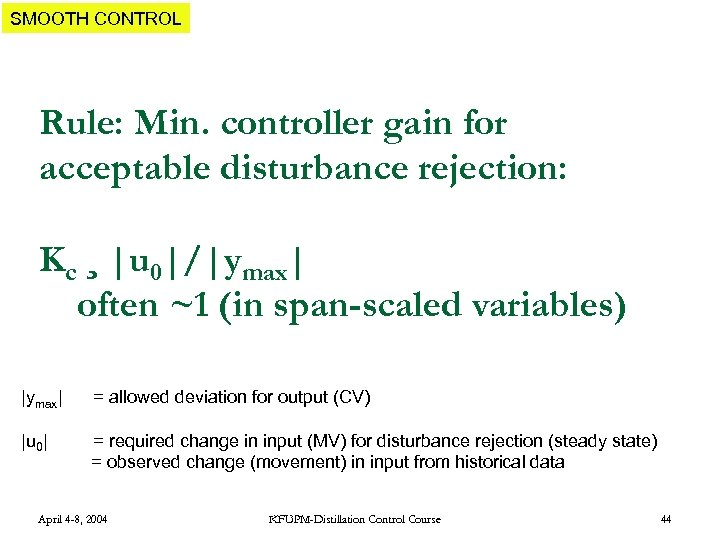SMOOTH CONTROL Rule: Min. controller gain for acceptable disturbance rejection: Kc ¸ |u 0|/|ymax| often ~1 (in span-scaled variables) |ymax| = allowed deviation for output (CV) |u 0| = required change in input (MV) for disturbance rejection (steady state) = observed change (movement) in input from historical data April 4 -8, 2004 KFUPM-Distillation Control Course 44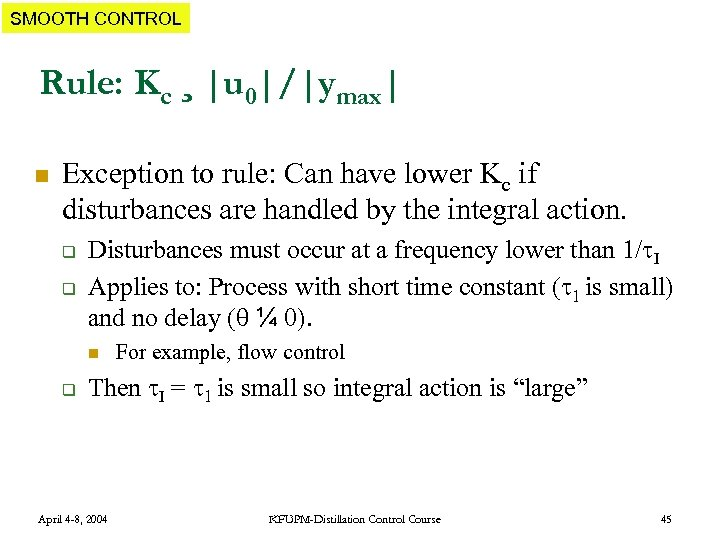SMOOTH CONTROL Rule: Kc ¸ |u 0|/|ymax| n Exception to rule: Can have lower Kc if disturbances are handled by the integral action. q q Disturbances must occur at a frequency lower than 1/ I Applies to: Process with short time constant ( 1 is small) and no delay ( ¼ 0). n q For example, flow control Then I = 1 is small so integral action is “large” April 4 -8, 2004 KFUPM-Distillation Control Course 45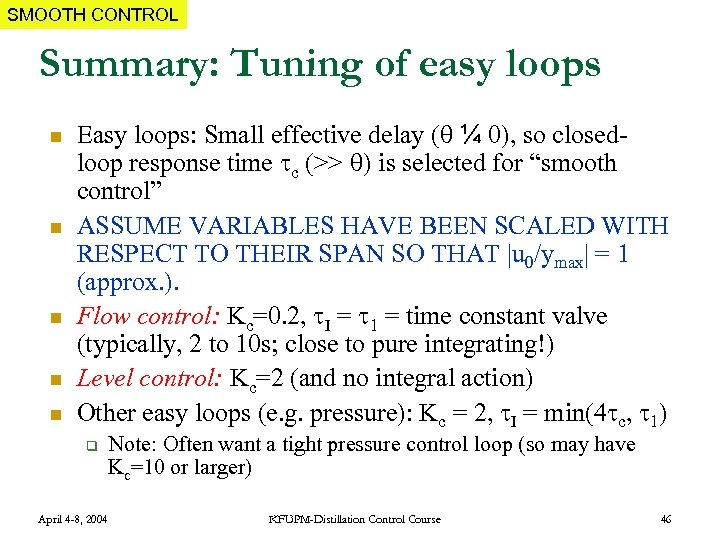SMOOTH CONTROL Summary: Tuning of easy loops n n n Easy loops: Small effective delay ( ¼ 0), so closedloop response time c (>> ) is selected for “smooth control” ASSUME VARIABLES HAVE BEEN SCALED WITH RESPECT TO THEIR SPAN SO THAT |u 0/ymax| = 1 (approx. ). Flow control: Kc=0. 2, I = 1 = time constant valve (typically, 2 to 10 s; close to pure integrating!) Level control: Kc=2 (and no integral action) Other easy loops (e. g. pressure): Kc = 2, I = min(4 c, 1) q April 4 -8, 2004 Note: Often want a tight pressure control loop (so may have Kc=10 or larger) KFUPM-Distillation Control Course 46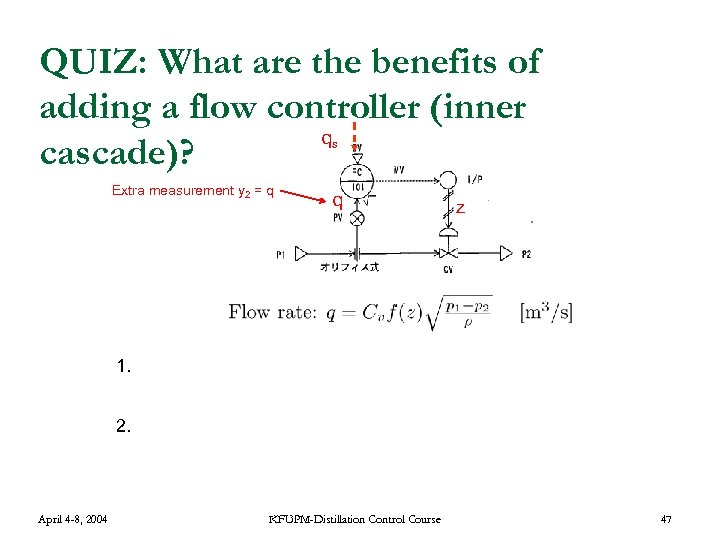QUIZ: What are the benefits of adding a flow controller (inner q cascade)? s Extra measurement y 2 = q 1. April 4 -8, 2004 z Counteracts nonlinearity in valve, f(z) • 2. q With fast flow control we can assume q = qs Eliminates effect of disturbances in p 1 and p 2 KFUPM-Distillation Control Course 47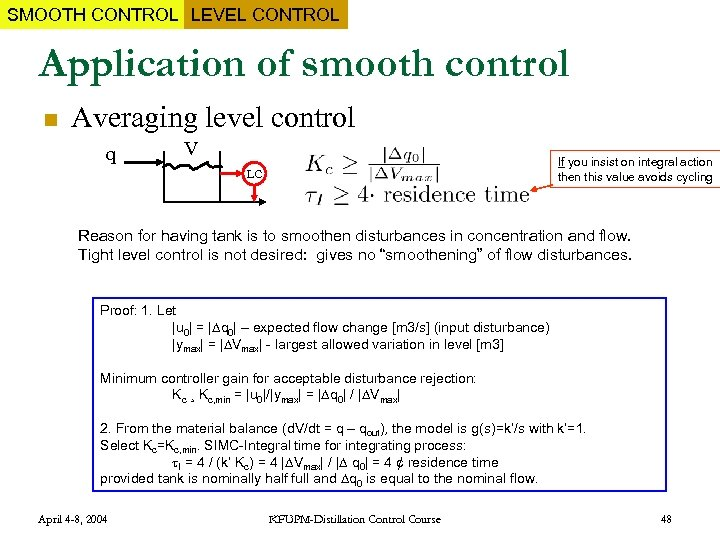SMOOTH CONTROL LEVEL CONTROL Application of smooth control n Averaging level control q V If you insist on integral action then this value avoids cycling LC Reason for having tank is to smoothen disturbances in concentration and flow. Tight level control is not desired: gives no “smoothening” of flow disturbances. Proof: 1. Let |u 0| = | q 0| – expected flow change [m 3/s] (input disturbance) |ymax| = | Vmax| - largest allowed variation in level [m 3] Minimum controller gain for acceptable disturbance rejection: Kc ¸ Kc, min = |u 0|/|ymax| = | q 0| / | Vmax| 2. From the material balance (d. V/dt = q – qout), the model is g(s)=k’/s with k’=1. Select Kc=Kc, min. SIMC-Integral time for integrating process: I = 4 / (k’ Kc) = 4 | Vmax| / | q 0| = 4 ¢ residence time provided tank is nominally half full and q 0 is equal to the nominal flow. April 4 -8, 2004 KFUPM-Distillation Control Course 48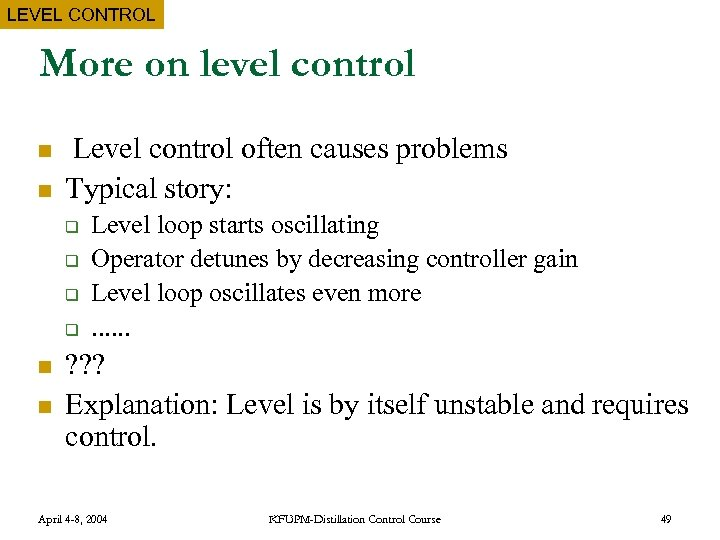LEVEL CONTROL More on level control n n Level control often causes problems Typical story: q q n n Level loop starts oscillating Operator detunes by decreasing controller gain Level loop oscillates even more. . . ? ? ? Explanation: Level is by itself unstable and requires control. April 4 -8, 2004 KFUPM-Distillation Control Course 49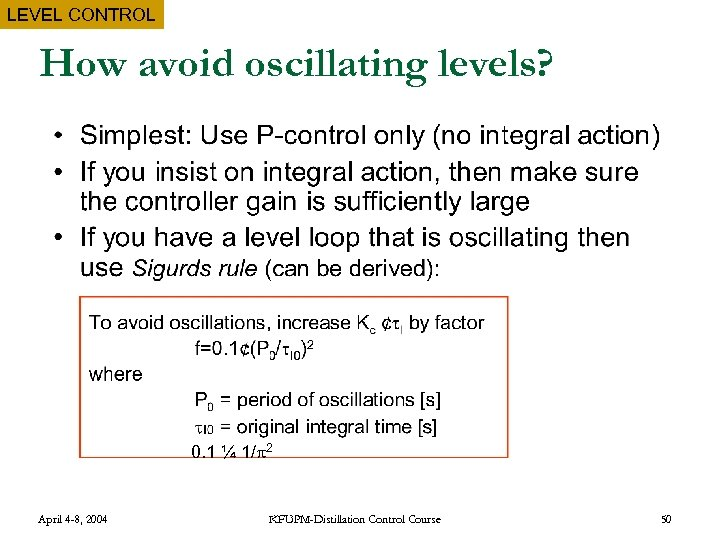LEVEL CONTROL How avoid oscillating levels? 0. 1 ¼ 1/ 2 April 4 -8, 2004 KFUPM-Distillation Control Course 50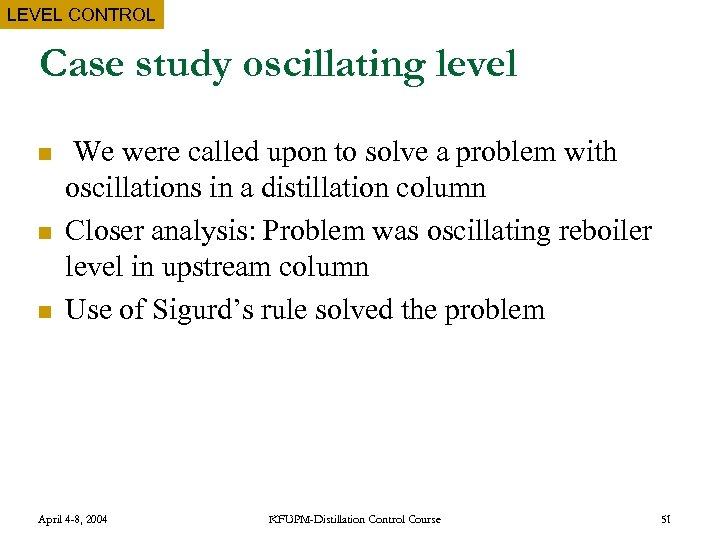LEVEL CONTROL Case study oscillating level n n n We were called upon to solve a problem with oscillations in a distillation column Closer analysis: Problem was oscillating reboiler level in upstream column Use of Sigurd’s rule solved the problem April 4 -8, 2004 KFUPM-Distillation Control Course 51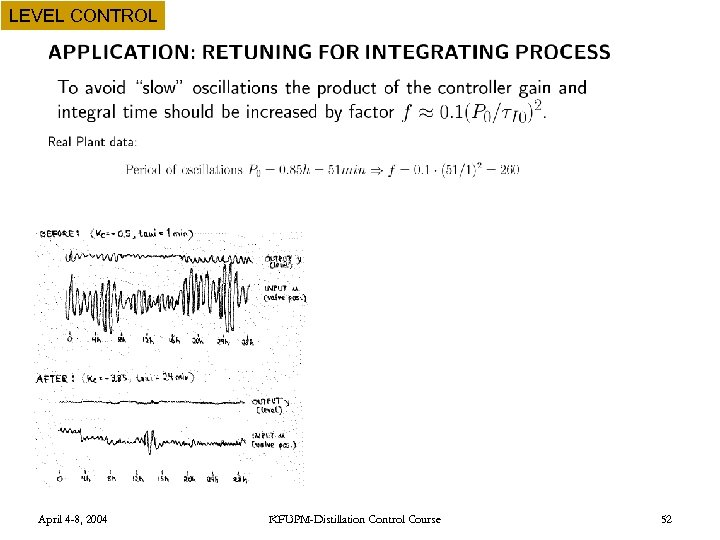LEVEL CONTROL April 4 -8, 2004 KFUPM-Distillation Control Course 52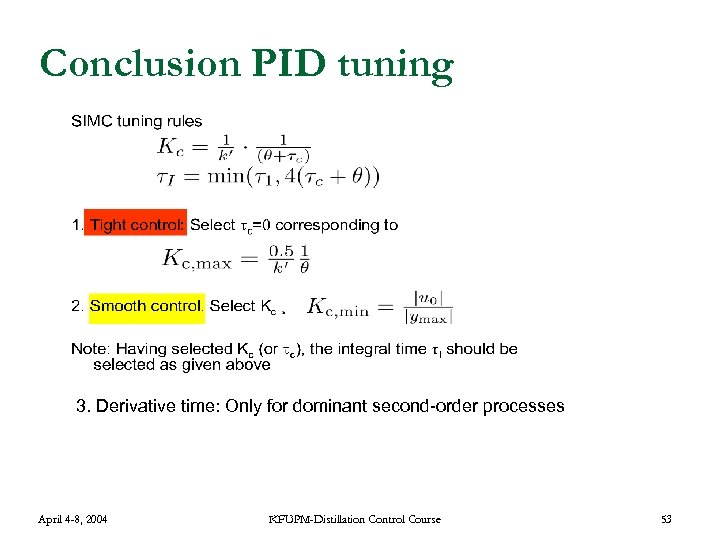Conclusion PID tuning 3. Derivative time: Only for dominant second-order processes April 4 -8, 2004 KFUPM-Distillation Control Course 53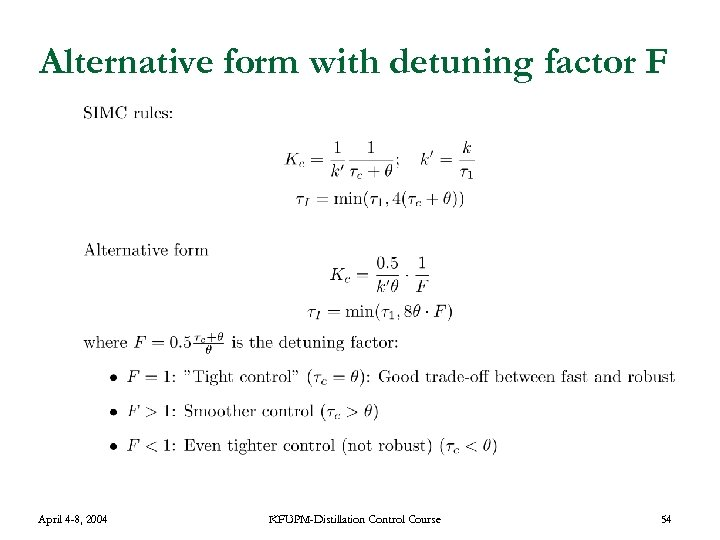Alternative form with detuning factor F April 4 -8, 2004 KFUPM-Distillation Control Course 54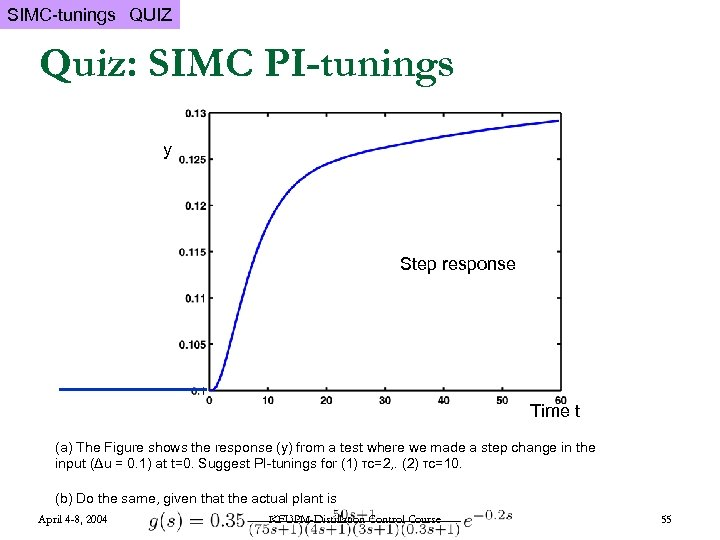SIMC-tunings QUIZ Quiz: SIMC PI-tunings y y Step response t [s]Time t (a) The Figure shows the response (y) from a test where we made a step change in the input (Δu = 0. 1) at t=0. Suggest PI-tunings for (1) τc=2, . (2) τc=10. (b) Do the same, given that the actual plant is April 4 -8, 2004 KFUPM-Distillation Control Course 55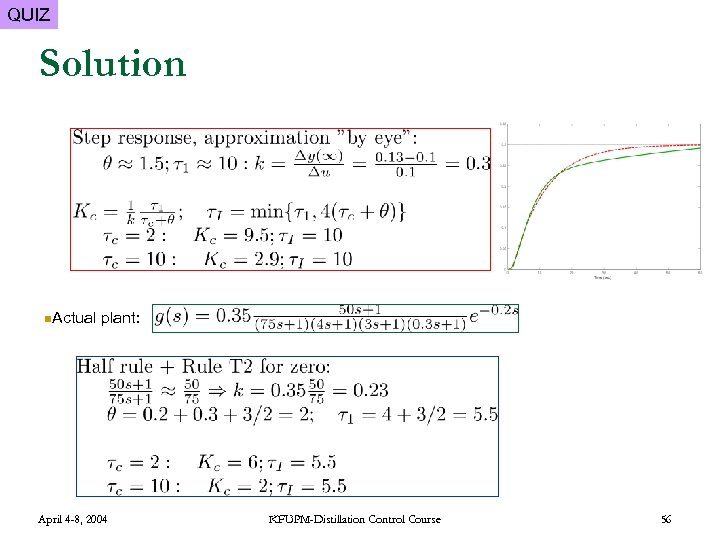QUIZ Solution n. Actual plant: April 4 -8, 2004 KFUPM-Distillation Control Course 56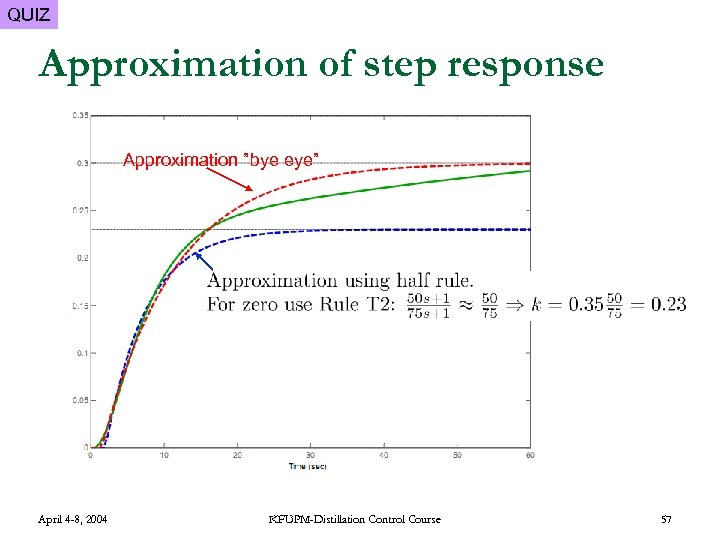QUIZ Approximation of step response Approximation ”bye eye” April 4 -8, 2004 KFUPM-Distillation Control Course 57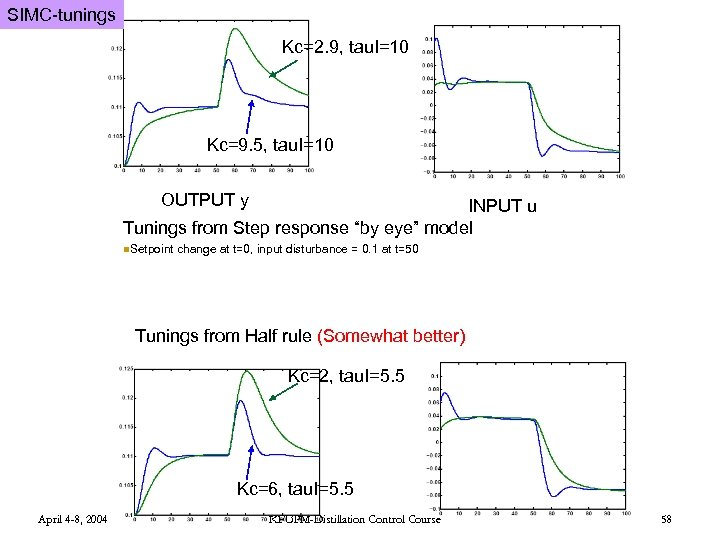SIMC-tunings Kc=2. 9, tau. I=10 Kc=9. 5, tau. I=10 OUTPUT y INPUT u Tunings from Step response “by eye” model n. Setpoint change at t=0, input disturbance = 0. 1 at t=50 Tunings from Half rule (Somewhat better) Kc=2, tau. I=5. 5 Kc=6, tau. I=5. 5 April 4 -8, 2004 KFUPM-Distillation Control Course 58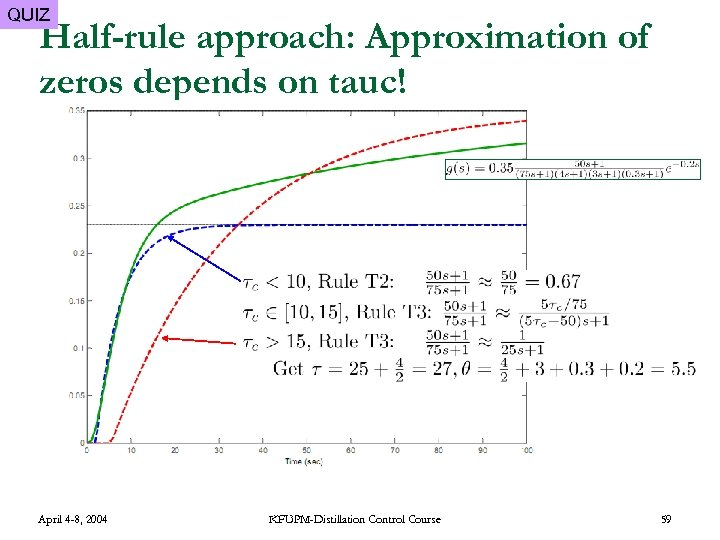QUIZ Half-rule approach: Approximation of zeros depends on tauc! April 4 -8, 2004 KFUPM-Distillation Control Course 59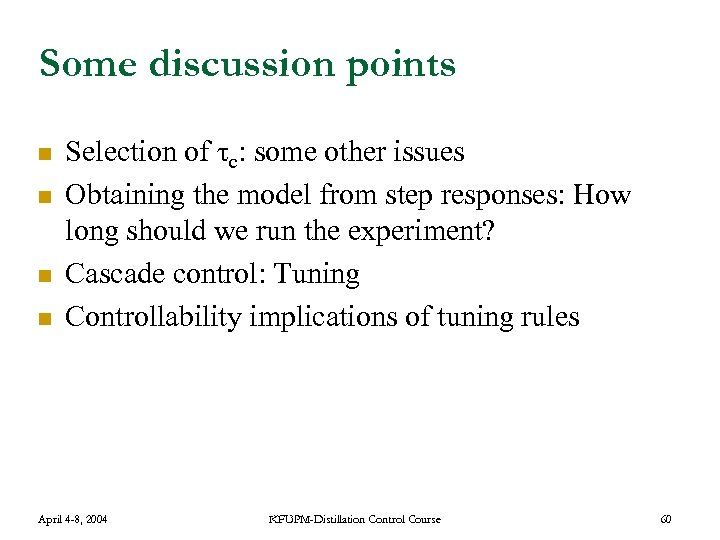Some discussion points n n Selection of τc: some other issues Obtaining the model from step responses: How long should we run the experiment? Cascade control: Tuning Controllability implications of tuning rules April 4 -8, 2004 KFUPM-Distillation Control Course 60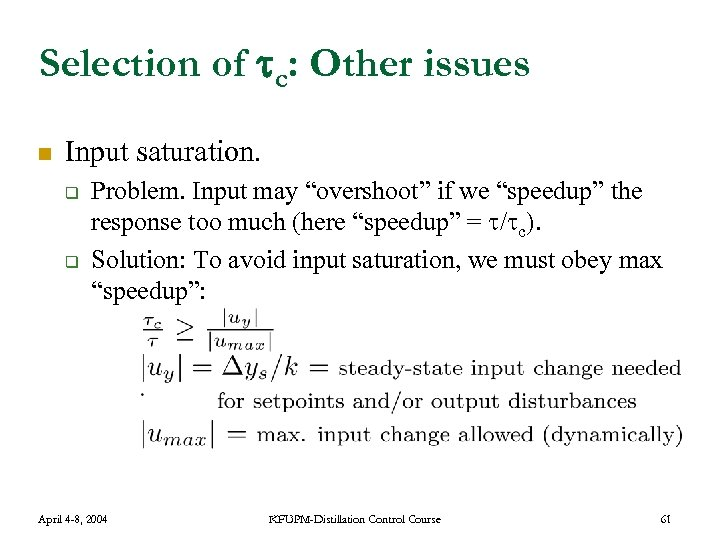Selection of c: Other issues n Input saturation. q q Problem. Input may “overshoot” if we “speedup” the response too much (here “speedup” = / c). Solution: To avoid input saturation, we must obey max “speedup”: April 4 -8, 2004 KFUPM-Distillation Control Course 61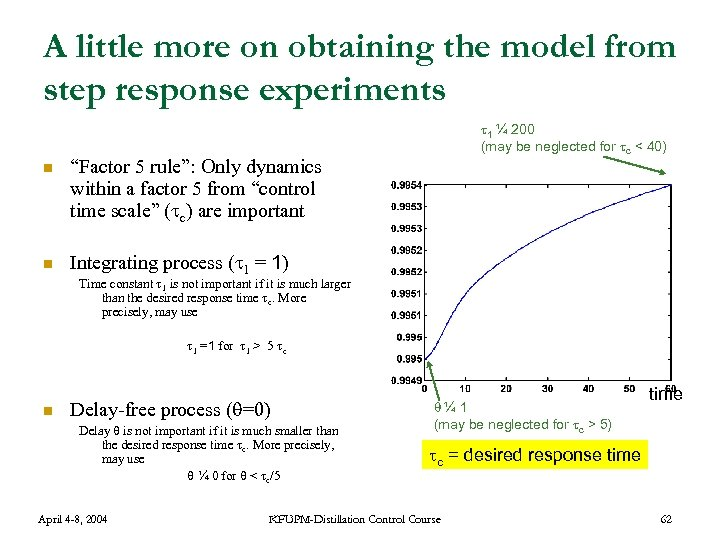A little more on obtaining the model from step response experiments n “Factor 5 rule”: Only dynamics within a factor 5 from “control time scale” ( c) are important n 1 ¼ 200 (may be neglected for c < 40) Integrating process ( 1 = 1) Time constant 1 is not important if it is much larger than the desired response time c. More precisely, may use 1 =1 for 1 > 5 c n Delay-free process ( =0) Delay is not important if it is much smaller than the desired response time c. More precisely, may use ¼ 0 for < c/5 April 4 -8, 2004 ¼ 1 (may be neglected for c > 5) time c = desired response time KFUPM-Distillation Control Course 62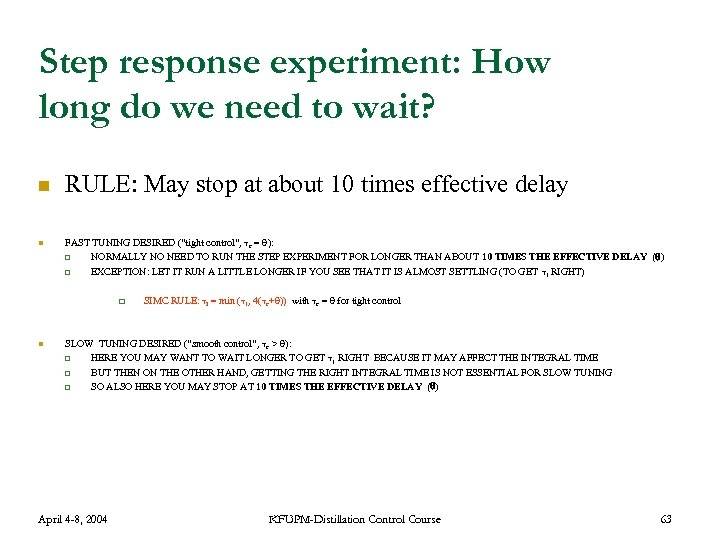Step response experiment: How long do we need to wait? n n RULE: May stop at about 10 times effective delay FAST TUNING DESIRED (“tight control”, c = ): q NORMALLY NO NEED TO RUN THE STEP EXPERIMENT FOR LONGER THAN ABOUT 10 TIMES THE EFFECTIVE DELAY ( ) q EXCEPTION: LET IT RUN A LITTLE LONGER IF YOU SEE THAT IT IS ALMOST SETTLING (TO GET 1 RIGHT) q n SIMC RULE: I = min ( 1, 4( c+ )) with c = for tight control SLOW TUNING DESIRED (“smooth control”, c > ): q HERE YOU MAY WANT TO WAIT LONGER TO GET 1 RIGHT BECAUSE IT MAY AFFECT THE INTEGRAL TIME q BUT THEN ON THE OTHER HAND, GETTING THE RIGHT INTEGRAL TIME IS NOT ESSENTIAL FOR SLOW TUNING q SO ALSO HERE YOU MAY STOP AT 10 TIMES THE EFFECTIVE DELAY ( ) April 4 -8, 2004 KFUPM-Distillation Control Course 63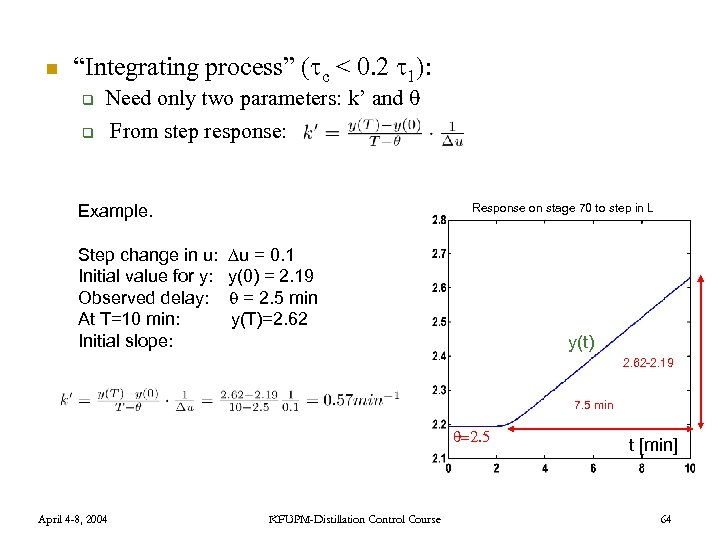n “Integrating process” ( c < 0. 2 1): q Need only two parameters: k’ and q From step response: Response on stage 70 to step in L Example. Step change in u: Initial value for y: Observed delay: At T=10 min: Initial slope: u = 0. 1 y(0) = 2. 19 = 2. 5 min y(T)=2. 62 y(t) 2. 62 -2. 19 7. 5 min =2. 5 April 4 -8, 2004 KFUPM-Distillation Control Course t [min] 64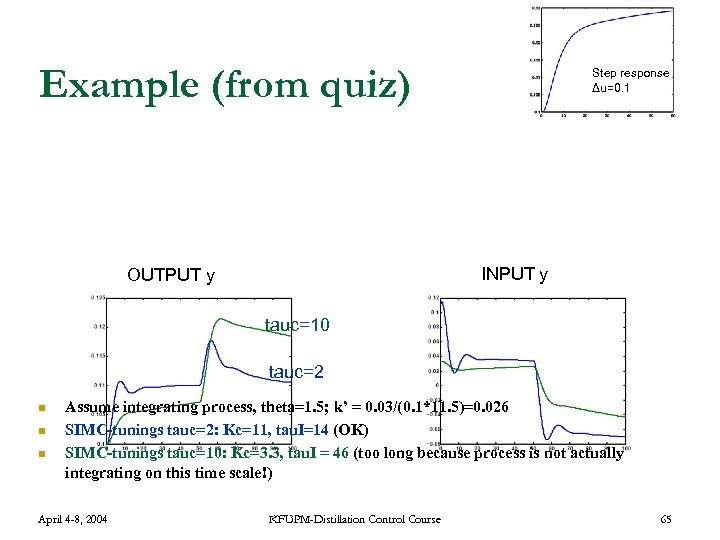Example (from quiz) Step response Δu=0. 1 INPUT y OUTPUT y tauc=10 tauc=2 n n n Assume integrating process, theta=1. 5; k’ = 0. 03/(0. 1*11. 5)=0. 026 SIMC-tunings tauc=2: Kc=11, tau. I=14 (OK) SIMC-tunings tauc=10: Kc=3. 3, tau. I = 46 (too long because process is not actually integrating on this time scale!) April 4 -8, 2004 KFUPM-Distillation Control Course 65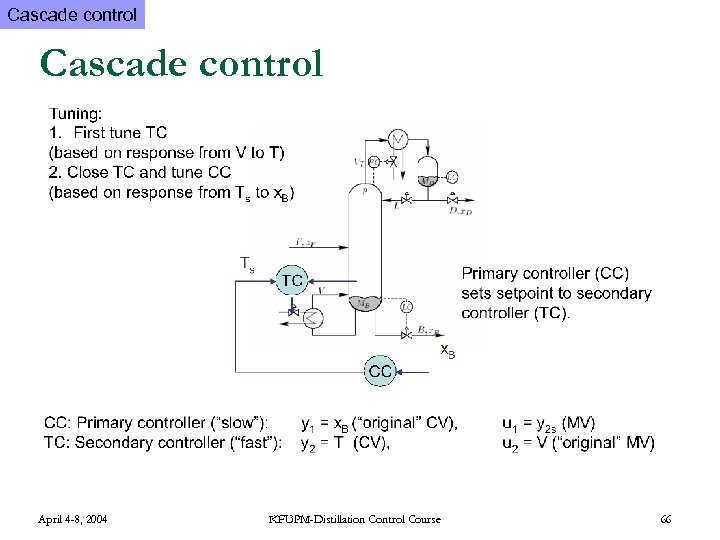Cascade control April 4 -8, 2004 KFUPM-Distillation Control Course 66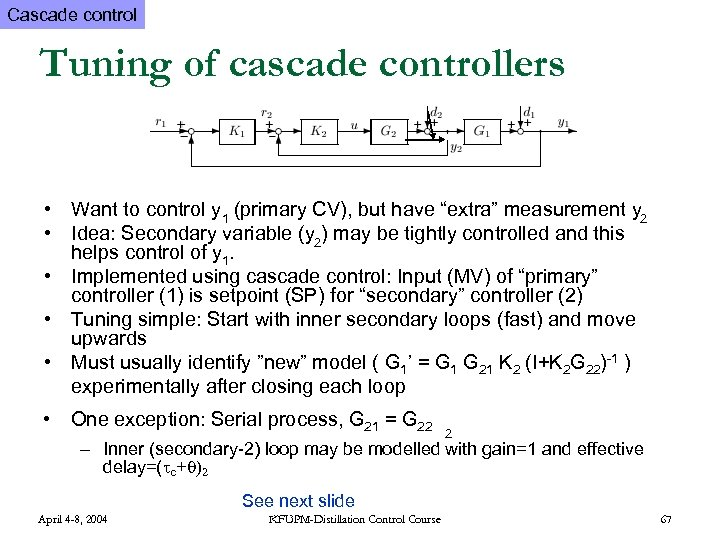Cascade control Tuning of cascade controllers • Want to control y 1 (primary CV), but have “extra” measurement y 2 • Idea: Secondary variable (y 2) may be tightly controlled and this helps control of y 1. • Implemented using cascade control: Input (MV) of “primary” controller (1) is setpoint (SP) for “secondary” controller (2) • Tuning simple: Start with inner secondary loops (fast) and move upwards • Must usually identify ”new” model ( G 1’ = G 1 G 21 K 2 (I+K 2 G 22)-1 ) experimentally after closing each loop • One exception: Serial process, G 21 = G 22 2 – Inner (secondary-2) loop may be modelled with gain=1 and effective delay=( c+ )2 See next slide April 4 -8, 2004 KFUPM-Distillation Control Course 67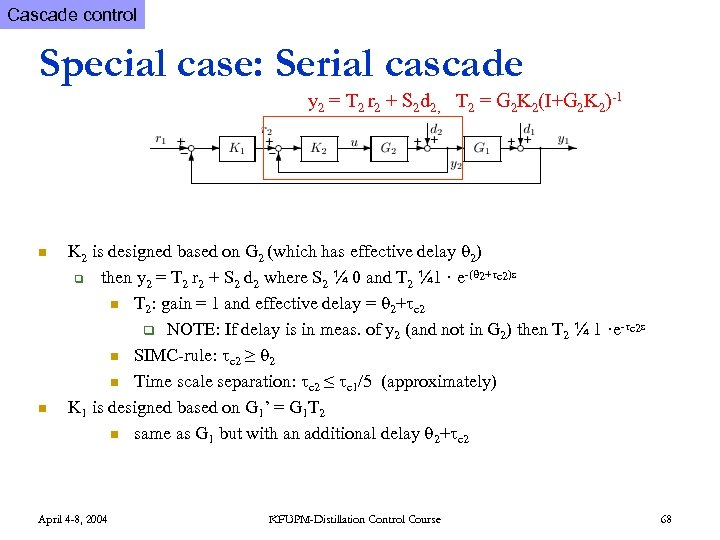Cascade control Special case: Serial cascade y 2 = T 2 r 2 + S 2 d 2, T 2 = G 2 K 2(I+G 2 K 2)-1 n n K 2 is designed based on G 2 (which has effective delay 2) q then y 2 = T 2 r 2 + S 2 d 2 where S 2 ¼ 0 and T 2 ¼ 1 · e-( 2+ c 2)s n T 2: gain = 1 and effective delay = 2+ c 2 q NOTE: If delay is in meas. of y 2 (and not in G 2) then T 2 ¼ 1 ·e- c 2 s n SIMC-rule: c 2 ≥ 2 n Time scale separation: c 2 ≤ c 1/5 (approximately) K 1 is designed based on G 1’ = G 1 T 2 n same as G 1 but with an additional delay 2+ c 2 April 4 -8, 2004 KFUPM-Distillation Control Course 68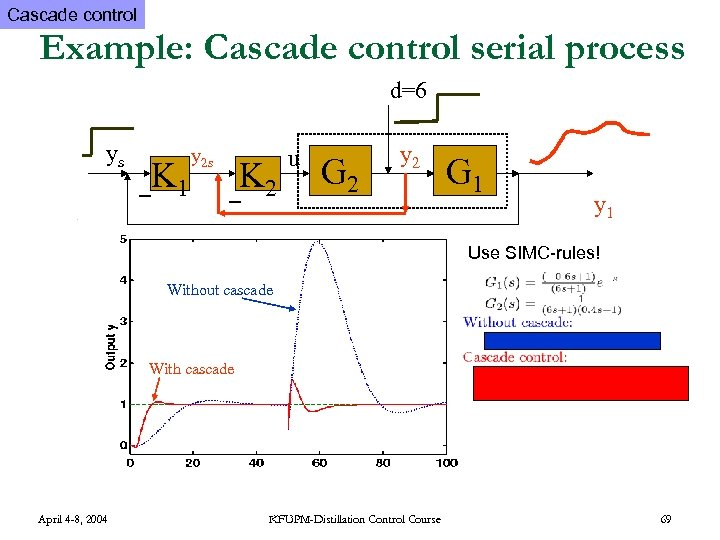Cascade control Example: Cascade control serial process d=6 ys K 1 y 2 s K 2 u G 2 y 2 G 1 y 1 Use SIMC-rules! Without cascade With cascade April 4 -8, 2004 KFUPM-Distillation Control Course 69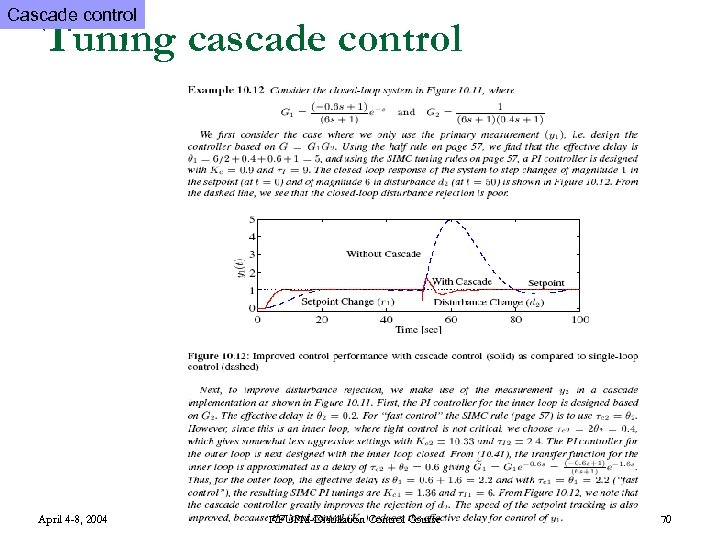Cascade control Tuning cascade control April 4 -8, 2004 KFUPM-Distillation Control Course 70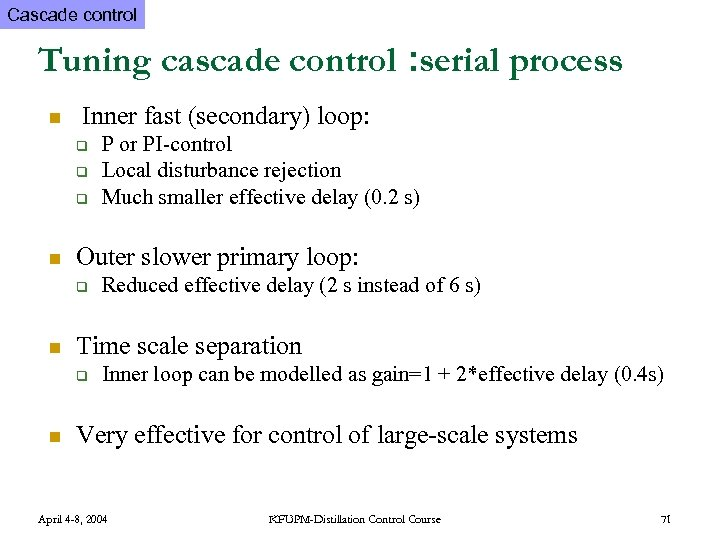Cascade control Tuning cascade control : serial process n Inner fast (secondary) loop: q q q n Outer slower primary loop: q n Reduced effective delay (2 s instead of 6 s) Time scale separation q n P or PI-control Local disturbance rejection Much smaller effective delay (0. 2 s) Inner loop can be modelled as gain=1 + 2*effective delay (0. 4 s) Very effective for control of large-scale systems April 4 -8, 2004 KFUPM-Distillation Control Course 71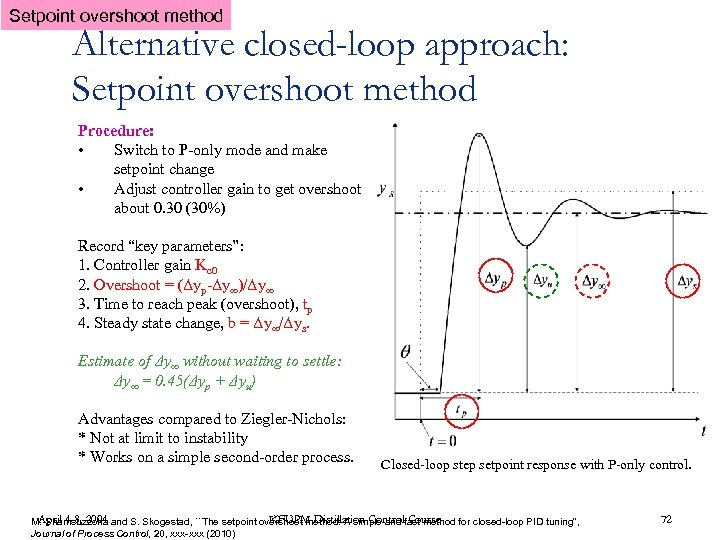Setpoint overshoot method Alternative closed-loop approach: Setpoint overshoot method Procedure: • Switch to P-only mode and make setpoint change • Adjust controller gain to get overshoot about 0. 30 (30%) Record “key parameters”: 1. Controller gain Kc 0 2. Overshoot = (Δyp-Δy∞)/Δy∞ 3. Time to reach peak (overshoot), tp 4. Steady state change, b = Δy∞/Δys. Estimate of Δy∞ without waiting to settle: Δy∞ = 0. 45(Δyp + Δyu) Advantages compared to Ziegler-Nichols: * Not at limit to instability * Works on a simple second-order process. Closed-loop step setpoint response with P-only control. April 4 -8, 2004 KFUPM-Distillation Control Course M. Shamsuzzoha and S. Skogestad, ``The setpoint overshoot method: A simple and fast method for closed-loop PID tuning'', Journal of Process Control, 20, xxx-xxx (2010) 72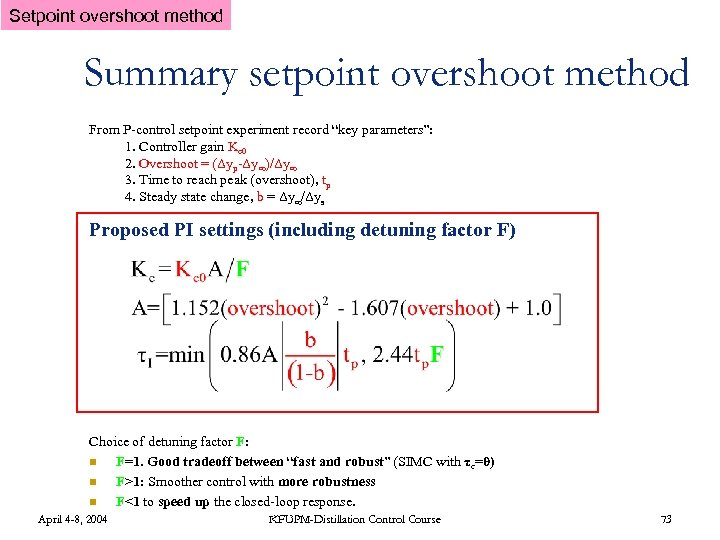Setpoint overshoot method Summary setpoint overshoot method From P-control setpoint experiment record “key parameters”: 1. Controller gain Kc 0 2. Overshoot = (Δyp-Δy∞)/Δy∞ 3. Time to reach peak (overshoot), tp 4. Steady state change, b = Δy∞/Δys Proposed PI settings (including detuning factor F) Choice of detuning factor F: n F=1. Good tradeoff between “fast and robust” (SIMC with τc=θ) n F>1: Smoother control with more robustness n F<1 to speed up the closed-loop response. April 4 -8, 2004 KFUPM-Distillation Control Course 73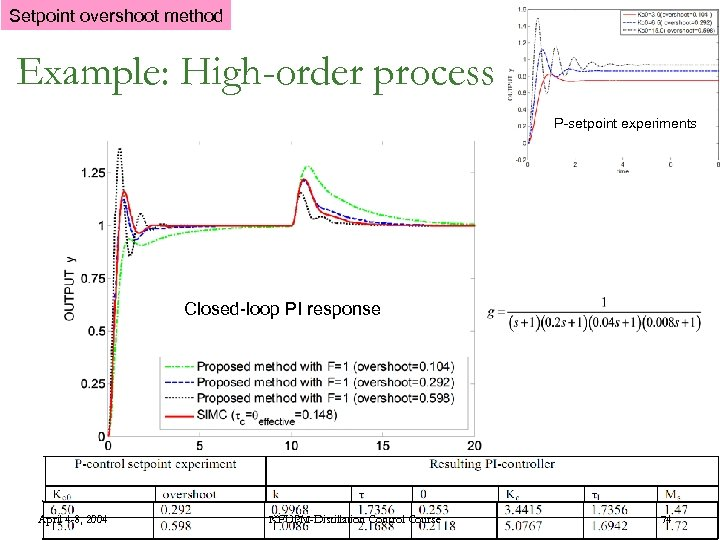Setpoint overshoot method Example: High-order process P-setpoint experiments Closed-loop PI response April 4 -8, 2004 KFUPM-Distillation Control Course 74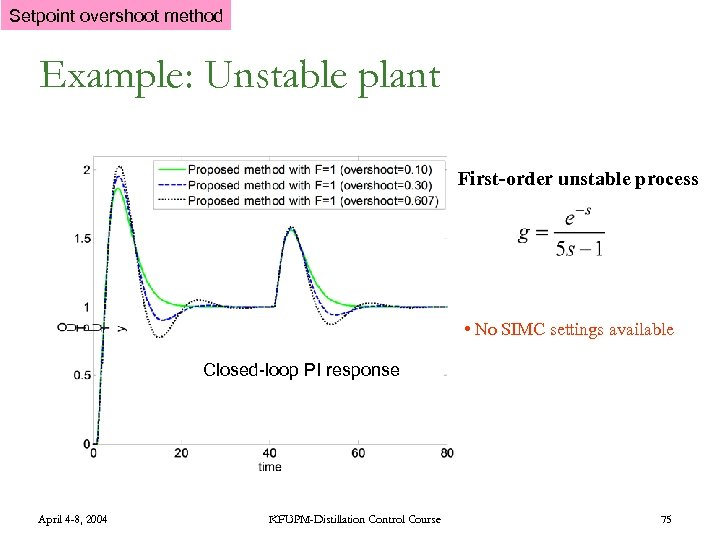Setpoint overshoot method Example: Unstable plant First-order unstable process • No SIMC settings available Closed-loop PI response April 4 -8, 2004 KFUPM-Distillation Control Course 75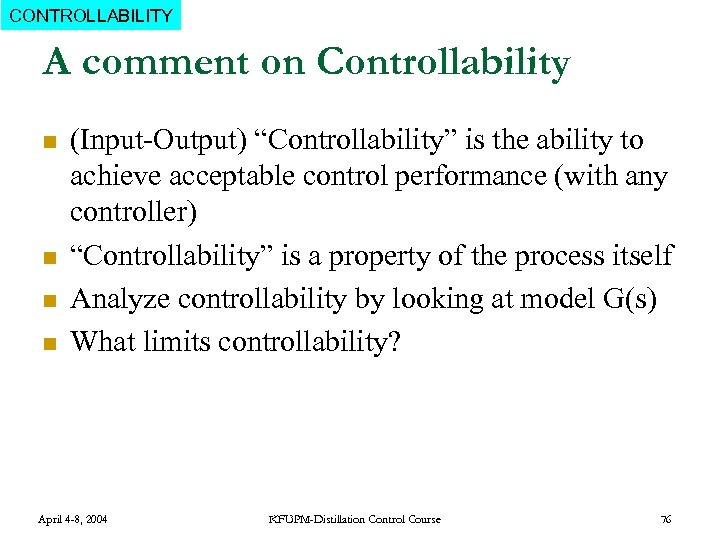CONTROLLABILITY A comment on Controllability n n (Input-Output) “Controllability” is the ability to achieve acceptable control performance (with any controller) “Controllability” is a property of the process itself Analyze controllability by looking at model G(s) What limits controllability? April 4 -8, 2004 KFUPM-Distillation Control Course 76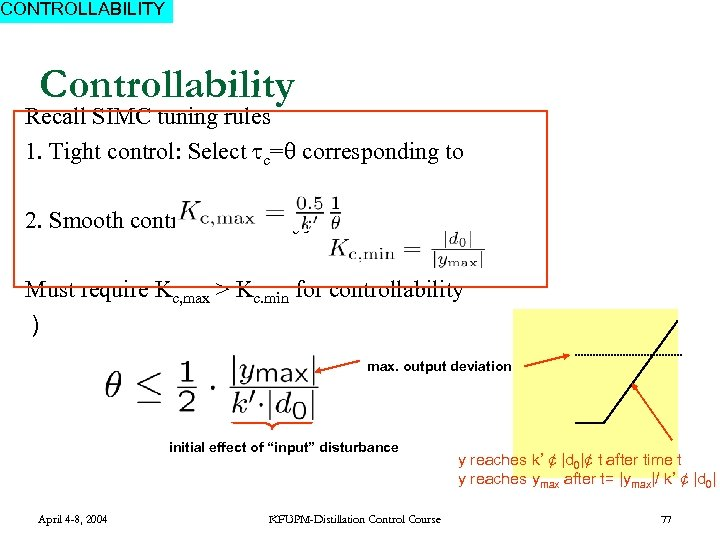CONTROLLABILITY Controllability Recall SIMC tuning rules 1. Tight control: Select c= corresponding to 2. Smooth control. Select Kc ¸ Must require Kc, max > Kc. min for controllability ) max. output deviation initial effect of “input” disturbance April 4 -8, 2004 KFUPM-Distillation Control Course y reaches k’ ¢ |d 0|¢ t after time t y reaches ymax after t= |ymax|/ k’ ¢ |d 0| 77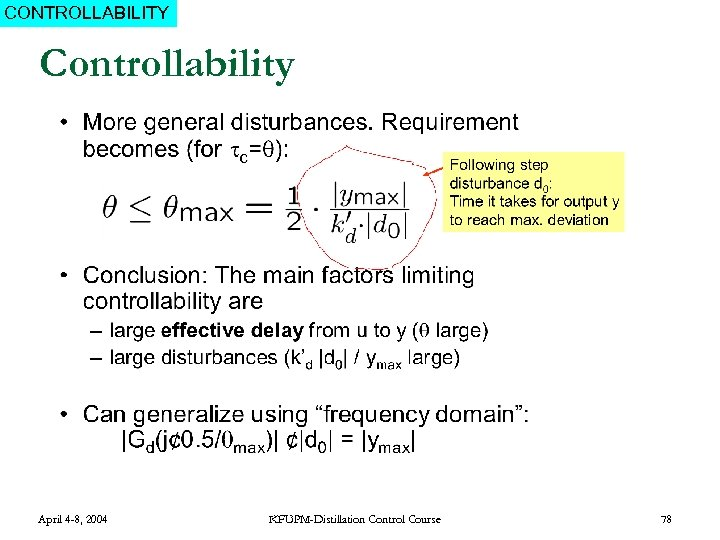CONTROLLABILITY Controllability April 4 -8, 2004 KFUPM-Distillation Control Course 78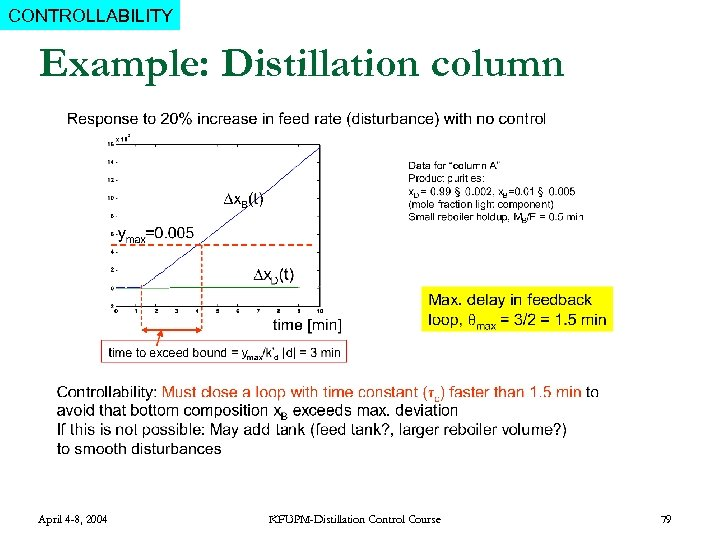CONTROLLABILITY Example: Distillation column April 4 -8, 2004 KFUPM-Distillation Control Course 79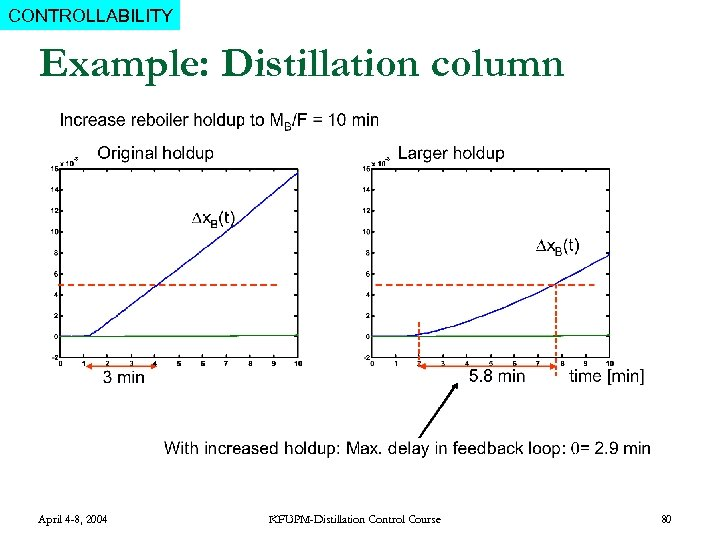CONTROLLABILITY Example: Distillation column April 4 -8, 2004 KFUPM-Distillation Control Course 80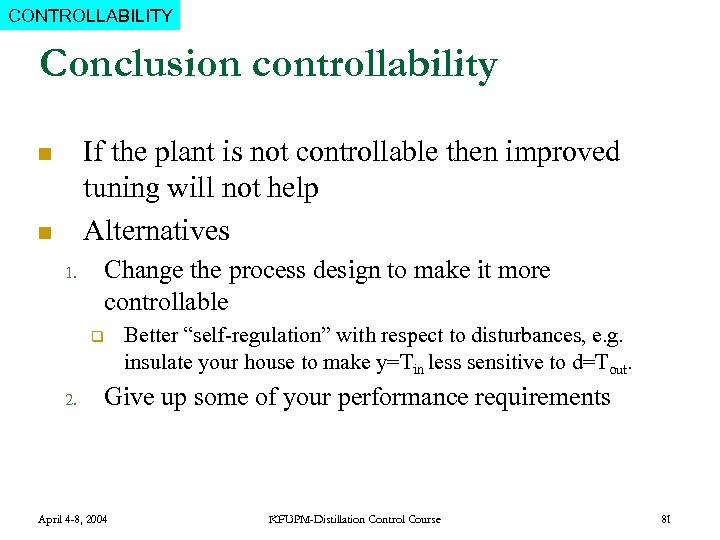CONTROLLABILITY Conclusion controllability If the plant is not controllable then improved tuning will not help Alternatives n n 1. Change the process design to make it more controllable q 2. Better “self-regulation” with respect to disturbances, e. g. insulate your house to make y=Tin less sensitive to d=Tout. Give up some of your performance requirements April 4 -8, 2004 KFUPM-Distillation Control Course 81